• 这里引入了常用降维算法模型原理，对MNIST 784维数据做可视化和结果对比展示，其中的大部分算法可用来减少数据维度，减少特征数，去除噪声干扰信息，压缩数据，降低计算量。公式原理等是引用他人内容，如侵权联系...
我们人类比较容易理解三维以内的信息，在做数据分析挖掘以前，先要对数据集有个浅显的认识，比如统计分布、可视化、相关性等。这里引入了常用降维算法模型原理，对MNIST 784维数据做可视化和结果对比展示，其中的大部分算法可用来减少数据维度，减少特征数，去除噪声干扰信息，压缩数据，降低计算量。公式原理等是引用他人内容，如侵权联系删除。
常用算法列举和特点
算法特点MNIST效果1. PCA线性降维、运行时间短(1s/3000条)极差TSNE非线性降维、运行时间短(1452s/70000条)一般LargeVis非线性降维、运行时间一般(686s/70000条)最好UMAP非线性降维、运行时间短(16s/4000条)较好AUTO_ENCODER非线性降维、运行时间一般(135s/65000条)一般

1. PCA
1.1 算法原理
PCA(Principal Component Analysis)，即主成分分析方法，是一种使用最广泛的数据线性降维算法。PCA的主要思想是将n维特征映射到k(其中k<n)维上，这k维是全新的正交特征也被称为主成分，是在原有n维特征的基础上重新构造出来的k维特征。PCA的工作就是从原始的空间中顺序地找一组相互正交的坐标轴，新的坐标轴的选择与数据本身是密切相关的。其中，第一个新坐标轴选择是原始数据中方差最大的方向，第二个新坐标轴选取是与第一个坐标轴正交的平面中使得方差最大的，第三个轴是与第1,2个轴正交的平面中方差最大的。依次类推，可以得到n个这样的坐标轴。(这部分操作类似决策树，先找到一个包含信息最多的切分点，在分离后的结果内找另一个最大的切分点。)通过这种方式获得的新的坐标轴，我们发现，大部分方差都包含在前面k个坐标轴中，后面的坐标轴所含的方差几乎为0。于是，我们可以忽略余下的坐标轴，只保留前面k个含有绝大部分方差的坐标轴。事实上，这相当于只保留包含绝大部分方差的维度特征，而忽略包含方差几乎为0的特征维度，实现对数据特征的降维处理。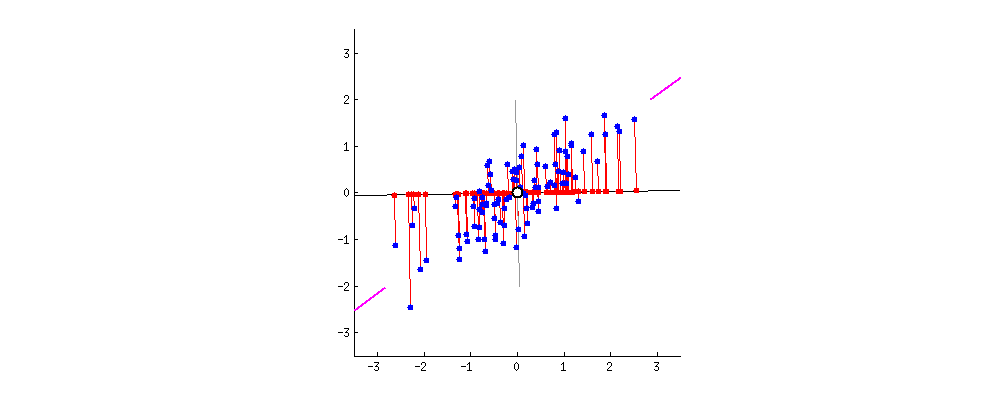1.1.1 协方差
数学定义协方差
提高计算效率我们先将特征均值化变为0，上面公式简化为
可以看到，在字段均值为0的情况下，两个字段的协方差简洁的表示为其内积除以元素数m。
当协方差为0时，表示两个字段完全独立。为了让协方差为0，我们选择第二个基时只能在与第一个基正交的方向上选择。因此最终选择的两个方向一定是正交的。
至此，我们得到了降维问题的优化目标：将一组N维向量降为K维（K大于0，小于N），其目标是选择K个单位（模为1）正交基，使得原始数据变换到这组基上后，各字段两两间协方差为0，而字段的方差则尽可能大（在正交的约束下，取最大的K个方差）。
矩阵是空间里的线性变换，PCA其实为矩阵分解，矩阵分解其实在原空间的子空间中找出线性变换中的主要变化趋势，减少冗余特征和不显著的特征。各维度相关性和噪声可以通过协方差矩阵表示，特征之间协方差为0去除了线性相关，剔除方差比较小的特征代表的噪声。
1.1.2 协方差矩阵
上面我们导出了优化目标，但是这个目标似乎不能直接作为操作指南（或者说算法），因为它只说要什么，但根本没有说怎么做。所以我们要继续在数学上研究计算方案。
我们看到，最终要达到的目的与字段内方差及字段间协方差有密切关系。因此我们希望能将两者统一表示，仔细观察发现，两者均可以表示为内积的形式，而内积又与矩阵相乘密切相关。于是我们来了灵感：
假设我们只有a和b两个字段，那么我们将它们按行组成矩阵X：

然后我们用X乘以X的转置，并乘上系数1/m：

奇迹出现了！这个矩阵对角线上的两个元素分别是两个字段的方差，而其它元素是a和b的协方差。两者被统一到了一个矩阵的。
根据矩阵相乘的运算法则，这个结论很容易被推广到一般情况：

设我们有m个n维数据记录，将其按列排成n乘m的矩阵X，设 ，则C是一个对称矩阵，其对角线分别为各个字段的方差，而第i行j列和j行i列元素相同，表示i和j两个字段的协方差。
1.1.3 协方差矩阵对角化
根据上述推导，我们发现要达到优化目标，等价于将协方差矩阵对角化：即除对角线外的其它元素化为0，并且在对角线上将元素按大小从上到下排列，这样我们就达到了优化目的。这样说可能还不是很明晰，我们进一步看下原矩阵与基变换后矩阵协方差矩阵的关系：
设原始数据矩阵X对应的协方差矩阵为C，而P是一组基按行组成的矩阵，设Y=XP，则Y为X对P做基变换后的数据。设Y的协方差矩阵为D，我们推导一下D与C的关系：

现在事情很明白了！我们要找的P不是别的，而是能让原始协方差矩阵对角化的P。换句话说，优化目标变成了寻找一个矩阵P，满足是一个对角矩阵，并且对角元素按从大到小依次排列，那么P的前K行就是要寻找的基，用P的前K行组成的矩阵乘以X就使得X从N维降到了K维并满足上述优化条件。
至此，我们离“发明”PCA还有仅一步之遥！
现在所有焦点都聚焦在了协方差矩阵对角化问题上，有时，我们真应该感谢数学家的先行，因为矩阵对角化在线性代数领域已经属于被玩烂了的东西，所以这在数学上根本不是问题。
由上文知道，协方差矩阵C是一个是对称矩阵，在线性代数上，实对称矩阵有一系列非常好的性质：
1）实对称矩阵不同特征值对应的特征向量必然正交。
2）设特征向量λ重数为r，则必然存在r个线性无关的特征向量对应于λ，因此可以将这r个特征向量单位正交化。
由上面两条可知，一个n行n列的实对称矩阵一定可以找到n个单位正交特征向量，设这n个特征向量为
我们将其按列组成矩阵：

则对协方差矩阵C有如下结论：

其中Λ为对角矩阵，其对角元素为各特征向量对应的特征值（可能有重复）。
以上结论不再给出严格的数学证明，对证明感兴趣的朋友可以参考线性代数书籍关于“实对称矩阵对角化”的内容。
到这里，我们发现我们已经找到了需要的矩阵P：
P=E
P是协方差矩阵的特征向量单位化后按行排列出的矩阵，其中每一行都是C的一个特征向量。如果设P按照Λ中特征值的从大到小，将特征向量从上到下排列，则用P的前K行组成的矩阵乘以原始数据矩阵X，就得到了我们需要的降维后的数据矩阵Y。
至此我们完成了整个PCA的数学原理讨论。在下面的一节，我们将给出PCA的一个实例。

1.2 算法及实例
为了巩固上面的理论，我们在这一节给出一个具体的PCA实例。
1.2.1 PCA算法
总结一下PCA的算法步骤，设有m条n维数据：
将原始数据按列组成m行n列矩阵目标结果是低维数据表示将X的每一行（代表一个属性字段）进行零均值化，即减去这一行的均值求出协方差矩阵求出协方差矩阵的特征值及对应的特征向量将特征向量按对应特征值大小从上到下按行排列成矩阵，取前k行组成矩阵PY=XP即为降维到k维后的数据
1.2.2 代码和结果
import numpy as np

from sklearn.decomposition import PCA
from sklearn.model_selection import train_test_split
from keras.datasets import mnist

(x_train, y_train), (x_test, y_test) = mnist.load_data()
train_data = x_train.reshape((-1, 784))
test_data = x_test.reshape((-1, 784))
data = np.concatenate((train_data, test_data), axis=0)
label = np.concatenate((y_train, y_test), axis=0)

_, x_run, _, y_run = train_test_split(test_data, y_test, test_size = 0.3, random_state=159)
x_run = x_run.astype('float32') / 255.0

pca = PCA(n_components=2)
x_run = pca.fit_transform(x_run)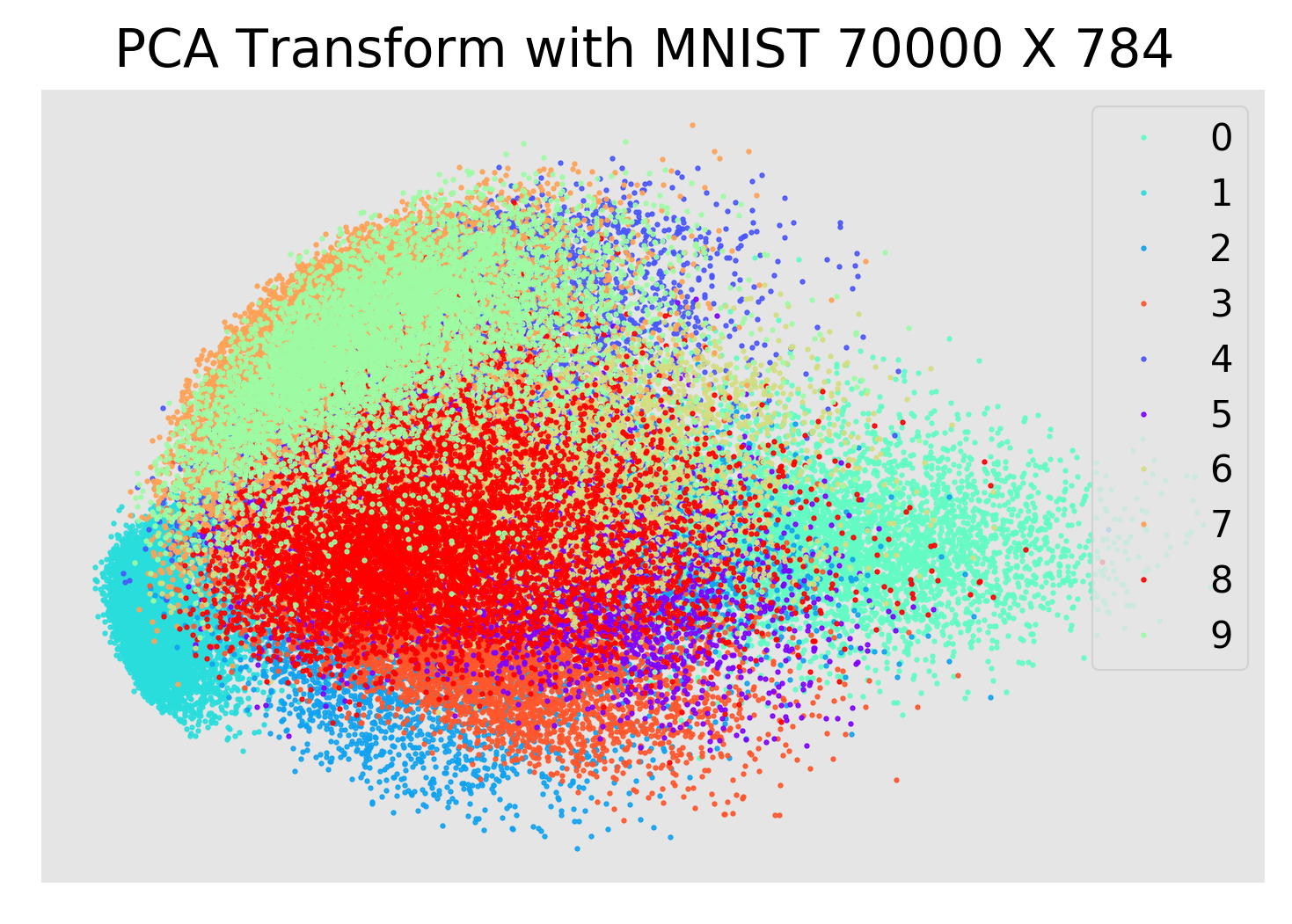2. TSNE
2.1 算法原理
t-SNE是由SNE(Stochastic Neighbor Embedding, SNE; Hinton and Roweis, 2002)发展而来。这里的Hinton就是大名鼎鼎的2018年图灵奖得主和深度学习的领跑者。
t-SNE(t-distributed stochastic neighbor embedding)是用于降维的一种机器学习算法，是由 Laurens van der Maaten 和 Geoffrey Hinton在08年提出来。此外，t-SNE 是一种非线性降维算法，非常适用于高维数据降维到2维或者3维，进行可视化。
我们先介绍SNE的基本原理，之后再扩展到t-SNE。最后再看一下t-SNE的实现以及一些优化。
2.1.1 流行学习
总觉得即使是“浅谈”两个字，还是让这个标题有些过大了，更何况我自己也才刚刚接触这么一个领域。不过懒得想其他标题了，想起来要扯一下这个话题，也是因为和朋友聊起我自己最近在做的方向。Manifold Learning 或者仅仅 Manifold 本身通常就听起来颇有些深奥的感觉，不过如果并不是想要进行严格的理论推导的话，也可以从许多直观的例子得到一些感性的认识，正好我也就借这个机会来简单地谈一下这个话题吧，或者说至少是我到目前为止对这它的认识。这两个词，在谈 Manifold 之前，不妨先说说 Learning ，也就是 Machine Learning 。而说道 Machine Learning 而不提一下 Artificial Intelligence 的话似乎又显得有些不厚道。人说 AI 是一门最悲剧的学科，因为每当它的一个子领域发展得像模像样之后，就立马自立门户，从此和 AI “再无瓜葛”了，而 Machine Learning 大概要算是最新的一个典型吧。这就让人有点奇怪，比如说数学，分门别类总算是够多了吧？可以不管怎么分，大家兄弟姐妹也都还承认自己是叫“数学”的。那 AI 呢？我觉得这里有很大一部分是它自身定位的问题。
反正现在我是不太清楚 AI 是做什么的，不知道其他人到底清楚不清楚。Wikipedia 上说
Artificial intelligence (AI) is the intelligence of machines and the branch of computer science that aims to create it.
可是这相当于一个 tautology ，因为到底什么又是 the intelligence of machines 呢？一开始的时候，大牛们都野心勃勃，而且好像也是信心满满，就好像曾经广泛认为“牛顿定理揭示了宇宙真理，科学剩下的事情只要按照公式来做计算就可以了”一样，大家可能觉得，不出几十年，人类就可以不用思考，交给 AI 来做了。不过我这里并不想再多说诸如什么是“思考”，什么是“智能”之类的以及随之而来的“图灵测试”之类的话题。我想说的是，到头来，AI 到底是什么，这还是一个问题，或者说，AI 在一开始定了一个过高的目标，几十年后，发现情况并不像当年那么乐观，却又有些下不了台了。
这个时候，AI 的一些旁枝或者子领域果断放下面子，丢掉了那个近乎玄幻的目标，逐渐发展成为“正常”的学科，所以也就不再好称为 AI 了。或者说现在的 AI 有两个意思，一个广义的 AI ，包括了所有相关的以及派生的领域，另一个则是狭义的或者经典的 AI ，专门指那些仍然在执着地追求着真正的“智能”的部分，或者说得不好听一点，就是剩下的部分。
Machine Learning 作为离家出走的典型，虽然名字里带了 Learning 一个词，让人乍一看觉得和 Intelligence 相比不过是换了个说法而已，然而事实上这里的 Learning 的意义要朴素得多。我们来看一看 Machine Learning 的典型的流程就知道了，其实有时候觉得和应用数学或者更通俗的数学建模有些类似，通常我们会有需要分析或者处理的数据，根据一些经验和一些假设，我们可以构建一个模型，这个模型会有一些参数（即使是非参数化方法，也是可以类似地看待的），根据数据来求解模型参数的过程，就叫做 Parameter Estimation ，或者 Model Fitting ，但是搞机器学习的人，通常把它叫做 Learning （或者，换一个角度，叫 Training）——因为根据数据归纳出一个有用的模型，这和我们人类“学习”的过程还是挺类似的吧。不过，如果抛开无聊的抠字眼游戏的话，我们可以看到，Machine Learning 已经抛弃了“智能”的高帽子，它的目的就是要解决具体的问题——而并不关心是否是通过一种“智能”的方式类解决的。
说到这里，其实我们构造模型就类似于写一个类，数据就是构造函数的参数，Learning 就是构造函数运行的过程，成功构造一个对象之后，我们就完成了学习。一些 Machine Learning 的问题到这一步就结束了，另一些情况还会使用得到的模型（对象）对后来的数据进行一些处理，通常会是 Inferencing 。到这个时候，又有些像统计里的东西了，所谓“统计推断”嘛。其实原本统计和机器学习研究的不少问题就是交叉在一起的，不过两派人从不同的角度来看待同样的问题。而且，也确实有 Statistical Learning 这么一个说法存在的，可以把他看成是 Machine Learning 的一个子领域（或者是一个分子或者甚至就是 Machine Learning 本身）。到这里，如果你还没有因为不断地抠字眼而烦躁的话，我已经忍无可忍了。所以，我就假定你已经了解了什么叫 Learning ，或者是已经恶心到懒得去了解了。于是我们转入下一个话题：流形，也就是 Manifold 。不知道你有没有为我在本文开头放上的那个地球的图片感到困惑？这是因为球面是一个很典型的流形的例子，而地球就是一个很典型的“球面”啦（姑且当作球面好啦）。
有时候经常会在 paper 里看到“嵌入在高维空间中的低维流形”，不过高维的数据对于我们这些可怜的低维生物来说总是很难以想像，所以最直观的例子通常都会是嵌入在三维空间中的二维或者一维流行。比如说一块布，可以把它看成一个二维平面，这是一个二维的欧氏空间，现在我们（在三维）中把它扭一扭，它就变成了一个流形（当然，不扭的时候，它也是一个流形，欧氏空间是流形的一种特殊情况）。
所以，直观上来讲，一个流形好比是一个 d 维的空间，在一个 m 维的空间中 (m > d) 被扭曲之后的结果。需要注意的是，流形并不是一个“形状”，而是一个“空间”，如果你觉得“扭曲的空间”难以想象，那么请再回忆之前一块布的例子。如果我没弄错的话，广义相对论似乎就是把我们的时空当作一个四维流（空间三维加上时间一维）形来研究的，引力就是这个流形扭曲的结果。当然，这些都是直观上的概念，其实流形并不需要依靠嵌入在一个“外围空间”而存在，稍微正式一点来说，一个 d 维的流形就是一个在任意点处局部同胚于（简单地说，就是正逆映射都是光滑的一一映射）欧氏空间。实际上，正是这种局部与欧氏空间的同胚给我们带来了很多好处，这使得我们在日常生活中许许多多的几何问题都可以使用简单的欧氏几何来解决，因为和地球的尺度比起来，我们的日常生活就算是一个很小的局部啦——我突然想起《七龙珠》里的那个界王住的那种私人小星球，走几步就要绕一圈的感觉，看来界王不仅要体力好（那上面重力似乎是地球的十倍），而且脑力也要好，初中学的必须是黎曼几何了！那么，除了地球这种简单的例子，实际应用中的数据，怎么知道它是不是一个流形呢？于是不妨又回归直观的感觉。再从球面说起，如果我们事先不知道球面的存在，那么球面上的点，其实就是三维欧氏空间上的点，可以用一个三元组来表示其坐标。但是和空间中的普通点不一样的是，它们允许出现的位置受到了一定的限制，具体到球面，可以可以看一下它的参数方程：

可以看到，这些三维的坐标实际上是由两个变量和生成的，也可以说成是它的自由度是二，也正好对应了它是一个二维的流形。有了这样的感觉之后，再来看流形学习里经常用到的人脸的例子，就很自然了。下图是 Isomap 论文里的一个结果：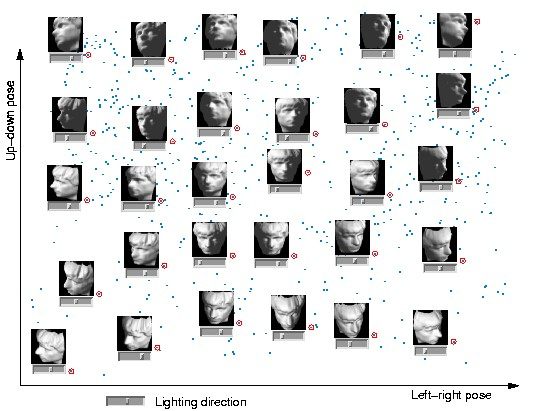这里的图片来自同一张人脸（好吧，其实是人脸模型），每张图片是 64×64 的灰度图，如果把位图按照列（或行）拼起来，就可以得到一个 4096 维的向量，这样一来，每一张图片就可以看成是 4096 维欧氏空间中的一个点。很显然，并不是 4096 维空间中任意一个点都可以对应于一张人脸图片的，这就类似于球面的情形，我们可以假定所有可以是人脸的 4096 维向量实际上分布在一个 d 维 (d < 4096) 的子空间中。而特定到 Isomap 的人脸这个例子，实际上我们知道所有的 698 张图片是拍自同一个人脸（模型），不过是在不同的 pose 和光照下拍摄的，如果把 pose （上下和左右）当作两个自由度，而光照当作一个自由度，那么这些图片实际只有三个自由度，换句话说，存在一个类似于球面一样的参数方程（当然，解析式是没法写出来的），给定一组参数（也就是上下、左右的 pose 和光照这三个值），就可以生成出对应的 4096 维的坐标来。换句话说，这是一个嵌入在 4096 维欧氏空间中的一个 3 维流形。 实际上，上面的那张图就是 Isomap 将这个数据集从 4096 维映射到 3 维空间中，并显示了其中 2 维的结果，图中的小点就是每个人脸在这个二维空间中对应的坐标位置，其中一些标红圈的点被选出来，并在旁边画上了该点对应的原始图片，可以很直观地看出这两个维度正好对应了 pose 的两个自由度平滑变化的结果。 就我目前所知，把流形引入到机器学习领域来主要有两种用途：一是将原来在欧氏空间中适用的算法加以改造，使得它工作在流形上，直接或间接地对流形的结构和性质加以利用；二是直接分析流形的结构，并试图将其映射到一个欧氏空间中，再在得到的结果上运用以前适用于欧氏空间的算法来进行学习。 这里 Isomap 正巧是一个非常典型的例子，因为它实际上是通过“改造一种原本适用于欧氏空间的算法”，达到了“将流形映射到一个欧氏空间”的目的。 :) Isomap 所改造的这个方法叫做 Multidimensional Scaling (MDS) ，MDS 是一种降维方法，它的目的就是使得降维之后的点两两之间的距离尽量不变（也就是和在原是空间中对应的两个点之间的距离要差不多）。只是 MDS 是针对欧氏空间设计的，对于距离的计算也是使用欧氏距离来完成的。如果数据分布在一个流形上的话，欧氏距离就不适用了。
让我们再回到地球——这个在三维空间中的二维流形，假设我们要在三维空间中计算北极点和南极点的距离，这很容易，就是两点相连的线段的长度，可是，如果要在这个流形上算距离就不能这样子算了，我们总不能从北极打个洞钻到南极去吧？要沿着地球表面走才行，当然，如果我随便沿着什么路线走一遍，然后数出总共走了多少步作为距离，这是不成的，因为这样一来如果我沿着不同的路线走，岂不是会得到不同的距离值？总而言之，我们现在需要一个新的定义在地球表面（流形）上的距离度量，理论上来说，任意满足测度的 4 个条件的函数都可以被定义为距离，不过，为了和欧氏空间对应起来，这里选择一个直线距离的推广定义。
还记得初中学的“两点之间，线段最短”吗？现在，我们反过来说，把线段的概念推广一下，变成“两点之间最短的曲线是线段”，于是流形上的距离定义也就等同于欧氏空间了：流形上两个点之间的距离就是连接两个点的“线段”的长度。虽然只是置换了一个概念，但是现在两者统一起来了，不过，在流形上的线段大概就不一定是“直”的了（于是直线也变成不一定是“直”的了），通常又称作是“测地线”。对于球面这个简单的流形来说，任意一条线段必定是在一个“大圆”上的，于是球面上的直线其实都是一些大圆，也造成了球面这个流形上没有平行线等一系列尴尬的局面（任意两条直线均相交），如果你看过一些数学科普八卦类的书，应该会回忆起不少东西啦！
回到 Isomap ，它主要做了一件事情，就是把 MDS 中原始空间中距离的计算从欧氏距离换为了流形上的测地距离。当然，如果流形的结构事先不知道的话，这个距离是没法算的，于是 Isomap 通过将数据点连接起来构成一个邻接 Graph 来离散地近似原来的流形，而测地距离也相应地通过 Graph 上的最短路径来近似了。如下图所示：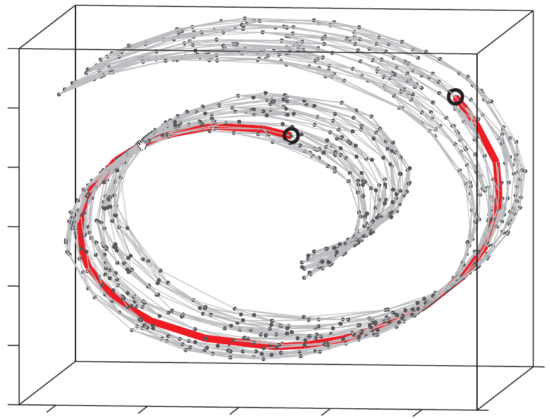这个东西叫做 Swiss Roll ，姑且把它看作一块卷起来的布好了。图中两个标黑圈的点，如果通过外围欧氏空间中的欧氏距离来计算的话，会是挨得很近的点，可是在流形上它们实际上是距离很远的点：红色的线是 Isomap 求出来的流形上的距离。可以想像，如果是原始的 MDS 的话，降维之后肯定会是很暴力地直接把它投影到二维空间中，完全无视流形结构，而 Isomap 则可以成功地将流形“展开”之后再做投影。
除了 Isomap 之外，Manifold Embedding 的算法还有很多很多，包括 Locally Linear Embedding 、Laplacian Eigenmaps 、Hessian Eigenmaps 、Local Tangent Space Alignment、Semidefinite Embedding (Maximum Variance Unfolding) 等等等等。哪个好哪个坏也不好说，它们都各有特点，而且也各自有不少变种。网上有一个 Matlab 的 demo ，给出了几种流行的 manifold embedding 算法在一些 synthetic manifold 上的结果和对比，可以有一个直观的认识。
另一方面是改造现有算法使其适合流形结构甚至专门针对流形的特点来设计新的算法，比较典型的是 graph regularized semi-supervised learning 。简单地说，在 supervised learning 中，我们只能利用有 label 的数据，而（通常都会有很多的）没有 label 的数据则白白浪费掉。在流形假设下，虽然这些数据没有 label ，但是仍然是可以有助于 Learn 出流形的结构的，而学出了流形结构之后实际上我们就是对原来的问题又多了一些认识，于是理所当然地期望能得到更好的结果喽。
当然，所有的这些都是基于同一个假设，那就是数据是分布在一个流形上的（部分算法可能会有稍微宽松一些的假设），然而 real world 的数据，究竟哪些是分别在流形上的呢？这个却是很难说。不过，除了典型的 face 和 hand written digit 之外，大家也有把基于流形的算法直接用在诸如 text 看起来好像也流形没有什么关系的数据上，效果似乎也还不错。
2.1.2 SNE原理
SNE是通过仿射(affinitie)变换将数据点映射到概率分布上，主要包括两个步骤：
SNE构建一个高维对象之间的概率分布，使得相似的对象有更高的概率被选择，而不相似的对象有较低的概率被选择。SNE在低维空间里在构建这些点的概率分布，使得这两个概率分布之间尽可能的相似。
我们看到t-SNE模型是非监督的降维，他跟kmeans等不同，他不能通过训练得到一些东西之后再用于其它数据（比如kmeans可以通过训练得到k个点，再用于其它数据集，而t-SNE只能单独的对数据做操作，也就是说他只有fit_transform，而没有fit操作）。
SNE是先将欧几里得距离转换为条件概率来表达点与点之间的相似度。具体来说，给定一个m个高维的数据（注意m不是维度）, t-SNE首先是计算概率,正比于和之间的相似度（这种概率是我们自主构建的），即：

这里的有一个参数是，对于不同的点取值不一样，后续会讨论如何设置。此外设置,因为我们关注的是两两之间的相似度。
那对于低维度下的，我们可以指定高斯分布为方差为，因此它们之间的相似度如下:

同样，设定.
如果降维的效果比较好，局部特征保留完整，那么, 因此我们优化两个分布之间的距离-KL散度(Kullback-Leibler divergences)，那么目标函数(cost function)如下:

这里的表示了给定点 下，其他所有数据点的条件概率分布。需要注意的是KL散度具有不对称性，在低维映射中不同的距离对应的惩罚权重是不同的，具体来说：距离较远的两个点来表达距离较近的两个点会产生更大的cost，相反，用较近的两个点来表达较远的两个点产生的cost相对较小(注意：类似于回归容易受异常值影响，但效果相反)。即用较小的来建模较大的, ,同样用较大的来建模较大的, cost=-0.277, 因此，SNE会倾向于保留数据中的局部特征。
下面我们提供一个参数的合理选择方式，首先不同的点具有不同的，的熵(entropy)会随着的增加而增加。SNE使用困惑度(perplexity)的概念，用二分搜索的方式来寻找一个最佳的σ。其中困惑度指:

这里的 是 的熵，即:

困惑度可以解释为一个点附近的有效近邻点个数。SNE对困惑度的调整比较有鲁棒性，通常选择5-50之间，给定之后，使用二分搜索的方式寻找合适的σ，那么核心问题是如何求解梯度了,目标函数等价于这个式子与softmax非常的类似，我们知道softmax的目标函数是，对应的梯度是(注：这里的softmax中y表示label，p表示预估值)。 同样我们可以推导SNE的目标函数中的i在j下的条件概率情况的梯度是， 同样j在i下的条件概率的梯度是, 最后得到完整的梯度公式如下:

在初始化中，可以用较小的σσ下的高斯分布来进行初始化。为了加速优化过程和避免陷入局部最优解，梯度中需要使用一个相对较大的动量(momentum)。即参数更新中除了当前的梯度，还要引入之前的梯度累加的指数衰减项，如下:

这里的表示迭代t次的解，表示学习速率,表示迭代t次的动量。
此外，在初始优化的阶段，每次迭代中可以引入一些高斯噪声，之后像模拟退火一样逐渐减小该噪声，可以用来避免陷入局部最优解。因此，SNE在选择高斯噪声，以及学习速率，什么时候开始衰减，动量选择等等超参数上，需要跑多次优化才可以。
2.1.3 TSNE原理
尽管SNE提供了很好的可视化方法，但是他很难优化，而且存在”crowding problem”(拥挤问题)。后续中，Hinton等人又提出了t-SNE的方法。与SNE不同，主要如下:
使用对称版的SNE，简化梯度公式低维空间下，使用t分布替代高斯分布表达两点之间的相似度
t-SNE在低维空间下使用更重长尾分布的t分布来避免crowding问题和优化问题。在这里，首先介绍一下对称版的SNE，之后介绍crowding问题，之后再介绍t-SNE。
优化和 的KL散度的一种替换思路是，使用联合概率分布来替换条件概率分布，即P是高维空间里各个点的联合概率分布，Q是低维空间下的，目标函数为:

这里的,为0，我们将这种SNE称之为symmetric SNE(对称SNE)，因为他假设了对于任意i,，因此概率分布可以改写为:

这种表达方式，使得整体简洁了很多。但是会引入异常值的问题。比如是异常值，那么会很大，对应的所有的j,
都会很小(之前是仅在下很小)，导致低维映射下的对cost影响很小。

为了解决这个问题，我们将联合概率分布定义修正为: , 这保证了, 使得每个点对于cost都会有一定的贡献。对称SNE的最大优点是梯度计算变得简单了，如下:

实验中，发现对称SNE能够产生和SNE一样好的结果，有时甚至略好一点。
优化后对称SNE降低了计算梯度复杂性，现在处理降维产生的拥挤问题。拥挤问题就是说各个簇聚集在一起，无法区分。比如有一种情况，高维度数据在降维到10维下，可以有很好的表达，但是降维到两维后无法得到可信映射，比如降维如10维中有11个点之间两两等距离的，在二维下就无法得到可信的映射结果(最多3个点)。 进一步的说明，假设一个以数据点为中心，半径为r的m维球(三维空间就是球)，其体积是按增长的，假设数据点是在m维球中均匀分布的，我们来看看其他数据点与的距离随维度增大而产生的变化。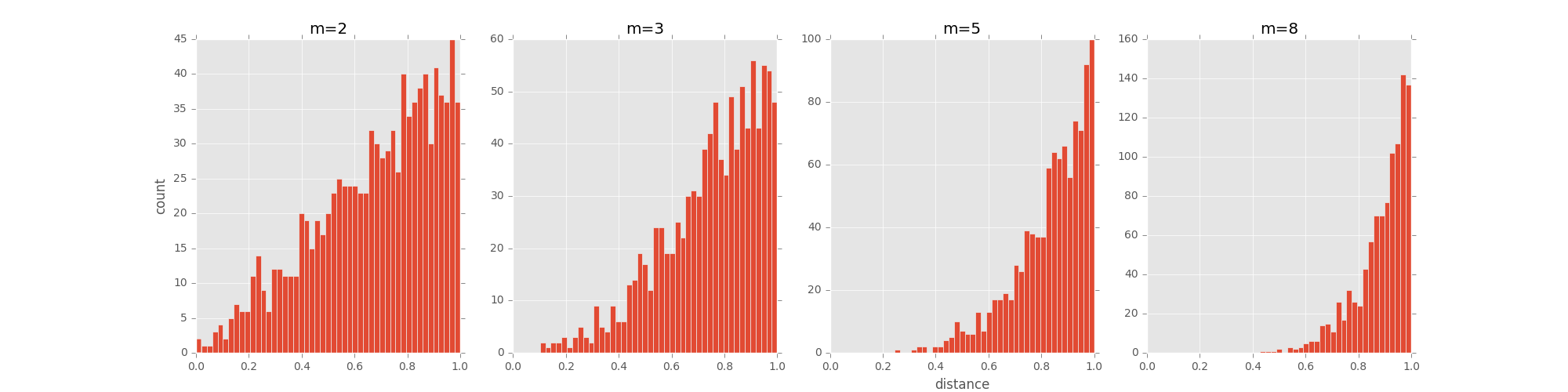从上图可以看到，随着维度的增大，大部分数据点都聚集在m维球的表面附近，与点的距离分布极不均衡。如果直接将这种距离关系保留到低维，就会出现拥挤问题。
两点间的距离公式，当维数增加一维要不原谅在根号内多增加了一项，比如两维，三维，维数上升至后距离变大了，逆操作降维距离变小。原来的高维距离较远的点会降维后距离变小，上图显示的是距离原点情况。
Cook et al.(2007) 提出一种slight repulsion的方式，在基线概率分布(uniform background)中引入一个较小的混合因子,这样就永远不会小于(因为一共了n(n-1)个pairs)，这样在高维空间中比较远的两个点之间的总是会比大一点。这种称之为UNI-SNE，效果通常比标准的SNE要好。优化UNI-SNE的方法是先让为0，使用标准的SNE优化，之后用模拟退火的方法的时候，再慢慢增加。直接优化UNI-SNE是不行的(即一开始不为0)，因为距离较远的两个点基本是一样的(等于基线分布), 即使很大，一些距离变化很难在中产生作用。也就是说优化中刚开始距离较远的两个聚类点，后续就无法再把他们拉近了。
对称SNE实际上在高维度下 另外一种减轻”拥挤问题”的方法：在高维空间下，在高维空间下我们使用高斯分布将距离转换为概率分布，在低维空间下，我们使用更加偏重长尾分布的方式来将距离转换为概率分布，使得高维度下中低等的距离在映射后能够有一个较大的距离。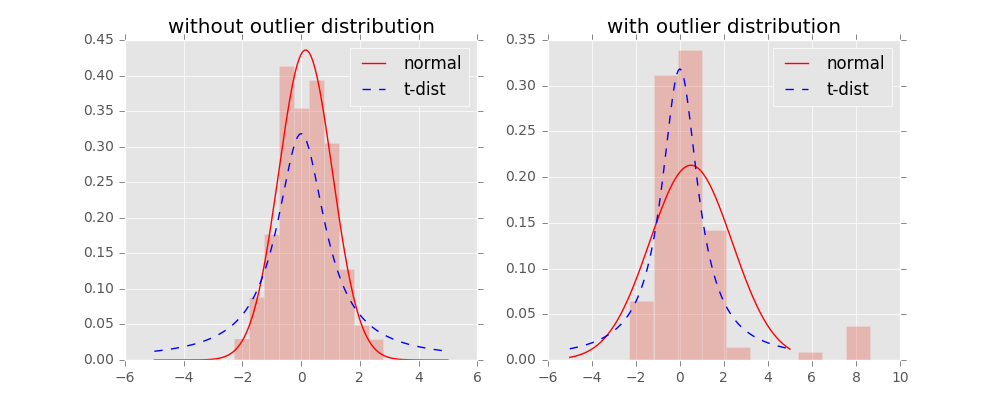我们对比一下高斯分布和t分布(如上图,code见probability/distribution.md), t分布受异常值影响更小，拟合结果更为合理，较好的捕获了数据的整体特征。
使用了t分布之后的q变化，如下:

此外，t分布是无限多个高斯分布的叠加，计算上不是指数的，会方便很多。优化的梯度如下: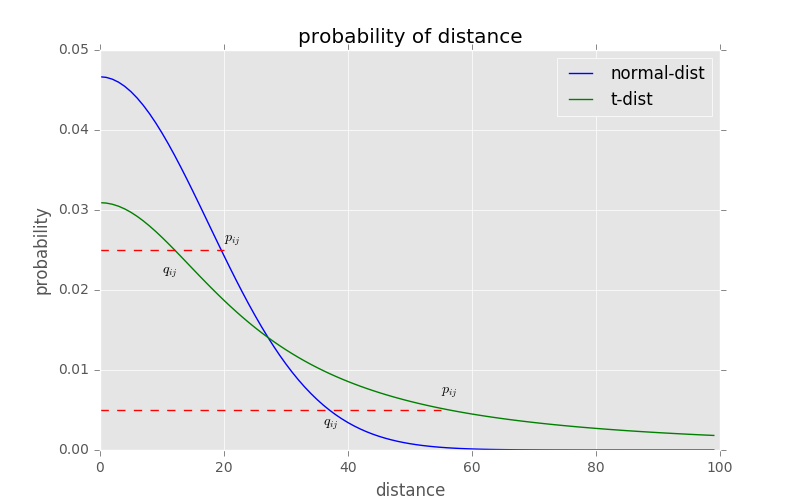t-sne的有效性，也可以从上图中看到：横轴表示距离，纵轴表示相似度, 可以看到，对于较大相似度的点，t分布在低维空间中的距离需要稍小一点；而对于低相似度的点，t分布在低维空间中的距离需要更远。这恰好满足了我们的需求，即同一簇内的点(距离较近)聚合的更紧密，不同簇之间的点(距离较远)更加疏远。
总结一下，t-SNE的梯度更新有两大优势：
对于不相似的点，用一个较小的距离会产生较大的梯度来让这些点排斥开来。这种排斥又不会无限大(梯度中分母)，避免不相似的点距离太远。

2.2 算法及实例
为了巩固上面的理论，我们在这一节给出一个具体的TSNE实例。
2.2.1 TSNE算法
总结一下TSNE的算法步骤，设有m条n维数据：
将原始数据按列组成m行n列矩阵计算cost function的参数：困惑度perplexity优化参数: 设置迭代次数T， 学习速率, 动量目标结果是低维数据表示开始优化

配合高维空间欧氏距离，在给定的perplexity下查找最佳使得，条件概率  令  用随机初始化 Y  从 t = 1 到 T迭代， 做如下操作:
计算低维空间的计算梯度更新
优化过程中可以尝试的两个trick:
提前压缩(early compression):开始初始化的时候，各个点要离得近一点。这样小的距离，方便各个聚类中心的移动。可以通过引入L2正则项(距离的平方和)来实现。提前夸大(early exaggeration)：在开始优化阶段，乘以一个大于1的数进行扩大，来避免因为太小导致优化太慢的问题。比如前50次迭代，乘以4
优化的过程动态图如下：2.2.2 代码和结果
import numpy as np

from sklearn.decomposition import PCA
from sklearn.manifold import TSNE
from sklearn.model_selection import train_test_split
from keras.datasets import mnist

(x_train, y_train), (x_test, y_test) = mnist.load_data()
train_data = x_train.reshape((-1, 784))
test_data = x_test.reshape((-1, 784))
data = np.concatenate((train_data, test_data), axis=0)
label = np.concatenate((y_train, y_test), axis=0)

_, x_run, _, y_run = train_test_split(test_data, y_test, test_size = 0.3, random_state=159)
x_run = x_run.astype('float32') / 255.0

pca = PCA(n_components=128)
x_run = pca.fit_transform(x_run)
components_list = [64, 2]
for dim in components_list:
tsne = TSNE(n_components=dim, perplexity=60, init='pca', verbose=1, random_state=0, method='exact')
x_run = tsne.fit_transform(x_run)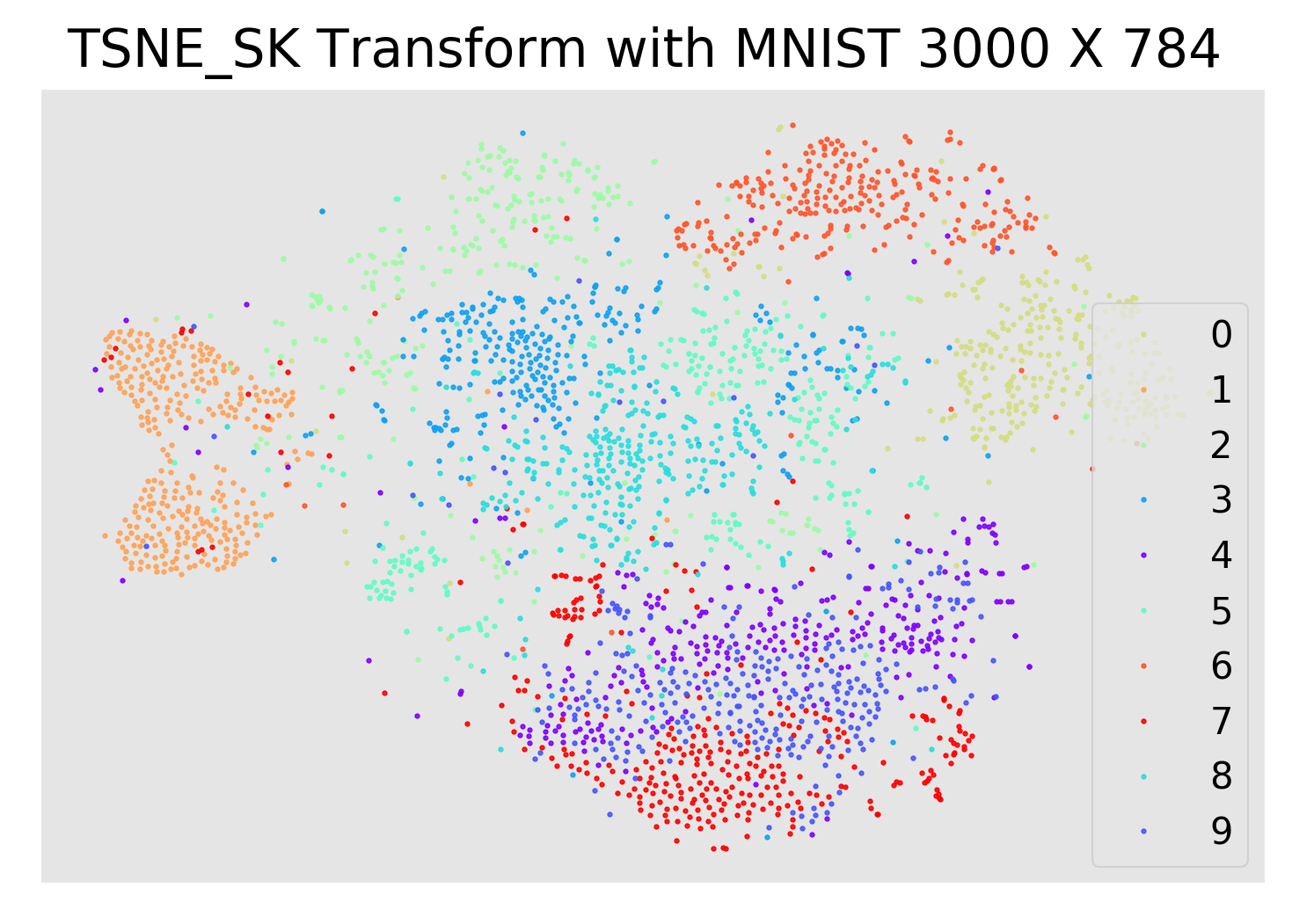3. LargeVis
3.1 算法及实例
3.1.1 代码和结果
import numpy as np

from keras.datasets import mnist

(x_train, y_train), (x_test, y_test) = mnist.load_data()
train_data = x_train.reshape((-1, 784))
test_data = x_test.reshape((-1, 784))
data = np.concatenate((train_data, test_data), axis=0)
label = np.concatenate((y_train, y_test), axis=0)

x_run = data.tolist()

x_run = LargeVis.run(2, 8, -1, -1, -1, -1, -1, -1, -1, -1)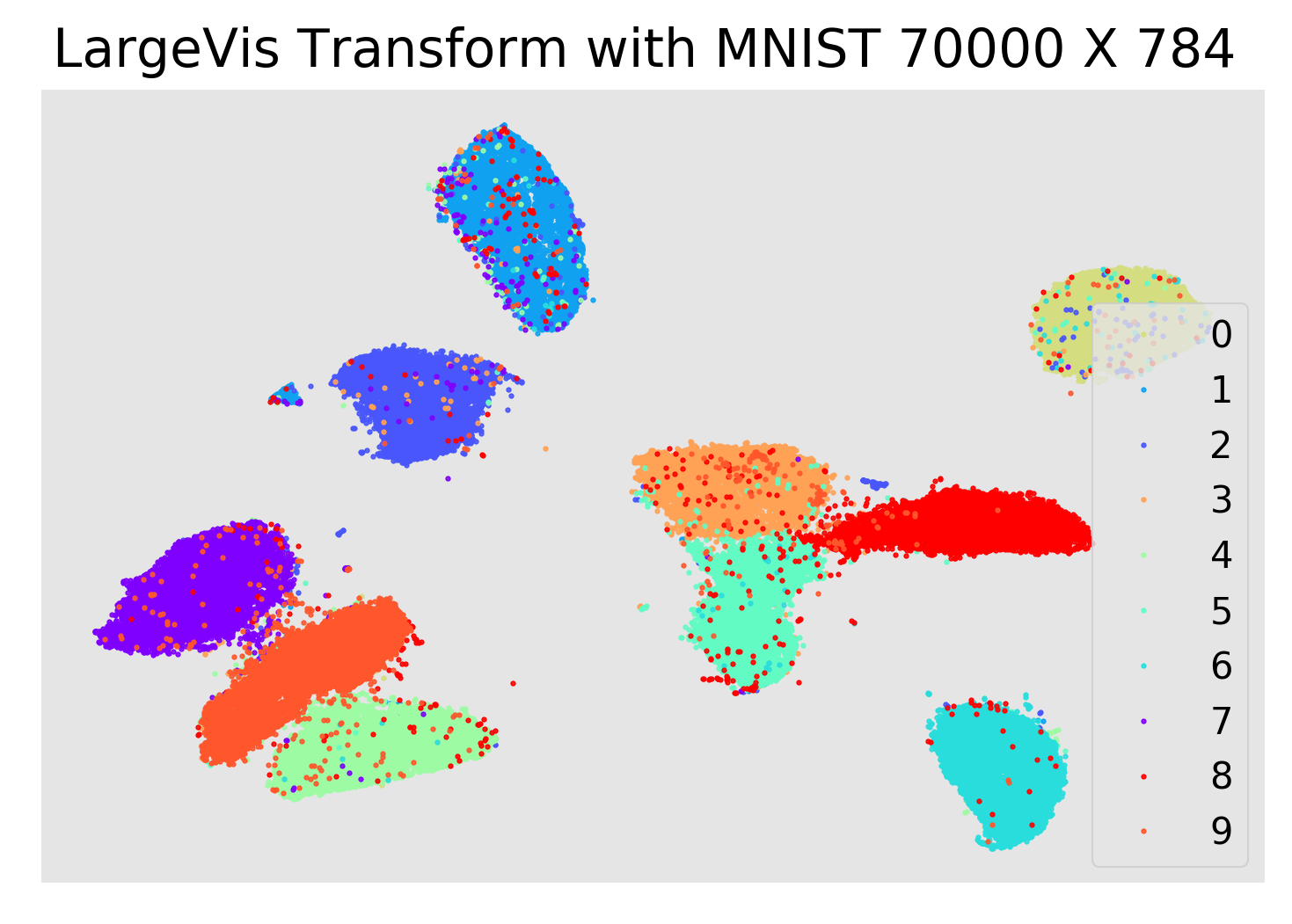4. UMAP
4.1 算法及实例
4.1.1 代码和结果
import numpy as np

from keras.datasets import mnist
from sklearn.decomposition import PCA
from sklearn.model_selection import train_test_split
import umap

(x_train, y_train), (x_test, y_test) = mnist.load_data()
train_data = x_train.reshape((-1, 784))
test_data = x_test.reshape((-1, 784))
data = np.concatenate((train_data, test_data), axis=0)
label = np.concatenate((y_train, y_test), axis=0)

_, x_run, _, y_run = train_test_split(test_data, y_test, test_size = 0.4, random_state=159)
x_run = x_run.astype('float32') / 255.0

pca = PCA(n_components=64)
x_run = pca.fit_transform(x_run)
x_run = umap.UMAP(n_neighbors=100, min_dist=0.5).fit_transform(x_run)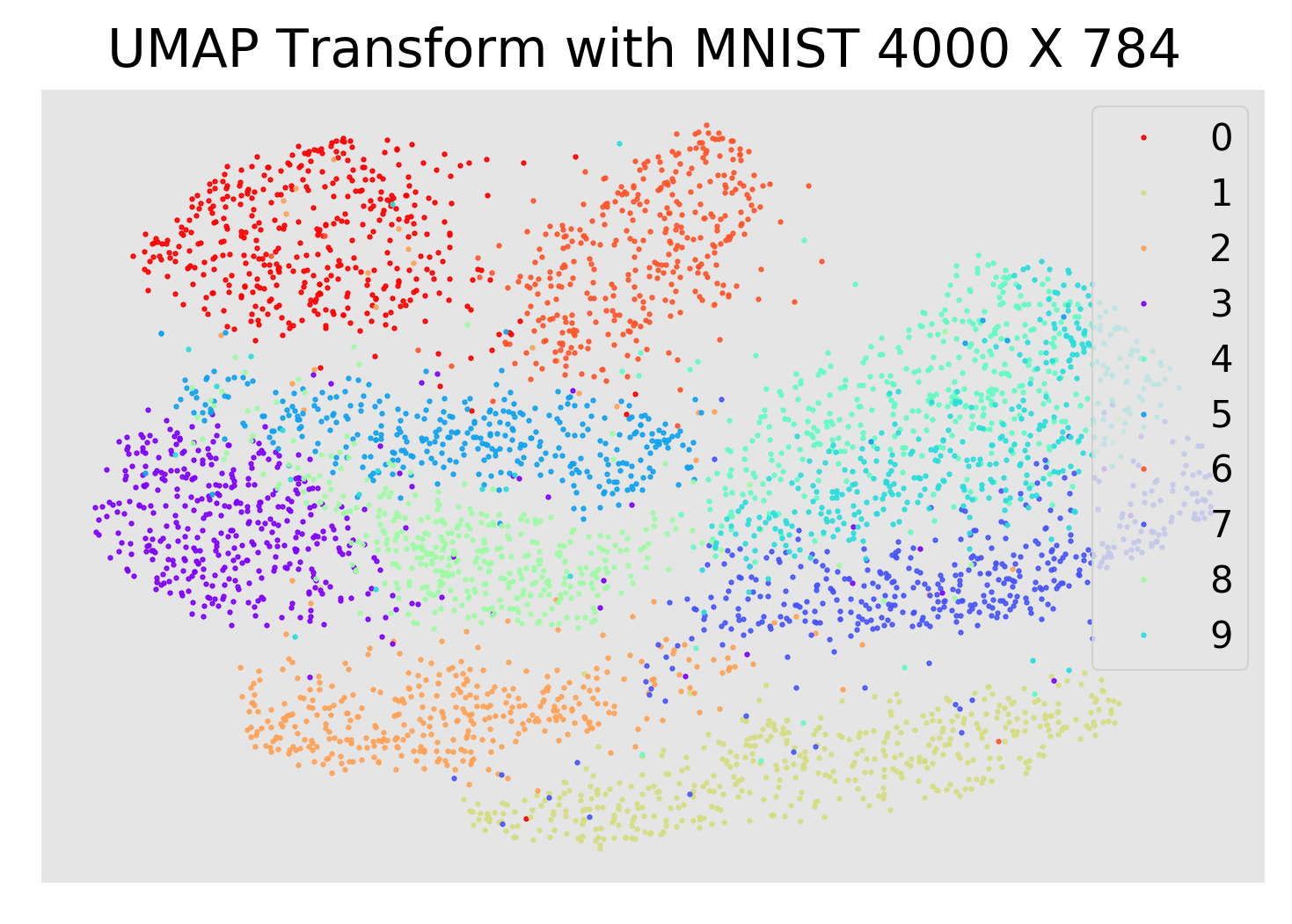5. AUTO_ENCODER
5.1 算法及实例
5.1.1 代码和结果
import tensorflow as tf
import numpy as np
from tensorflow.examples.tutorials.mnist import input_data

x_run = np.concatenate((mnist.train.images, mnist.test.images), axis=0)
y_run = np.concatenate((mnist.train.labels, mnist.test.labels), axis=0)

learning_rate = 0.01
training_epochs = 150
batch_size = 512
display_step = 1
num_samps, n_input = x_run.shape

X = tf.placeholder("float", [None, n_input])

# 用字典的方式存储各隐藏层的参数
n_hidden_1 = 128 # 第一编码层神经元个数
n_hidden_2 = 64 # 第二编码层神经元个数
n_hidden_3 = 10
n_hidden_4 = 2

# 权重和偏置的变化在编码层和解码层顺序是相逆的
# 权重参数矩阵维度是每层的 输入*输出，偏置参数维度取决于输出层的单元数
weights = {
'encoder_h1': tf.Variable(tf.truncated_normal([n_input, n_hidden_1], )),
'encoder_h2': tf.Variable(tf.truncated_normal([n_hidden_1, n_hidden_2], )),
'encoder_h3': tf.Variable(tf.truncated_normal([n_hidden_2, n_hidden_3], )),
'encoder_h4': tf.Variable(tf.truncated_normal([n_hidden_3, n_hidden_4], )),
'decoder_h1': tf.Variable(tf.truncated_normal([n_hidden_4, n_hidden_3], )),
'decoder_h2': tf.Variable(tf.truncated_normal([n_hidden_3, n_hidden_2], )),
'decoder_h3': tf.Variable(tf.truncated_normal([n_hidden_2, n_hidden_1], )),
'decoder_h4': tf.Variable(tf.truncated_normal([n_hidden_1, n_input], )),
}
biases = {
'encoder_b1': tf.Variable(tf.random_normal([n_hidden_1])),
'encoder_b2': tf.Variable(tf.random_normal([n_hidden_2])),
'encoder_b3': tf.Variable(tf.random_normal([n_hidden_3])),
'encoder_b4': tf.Variable(tf.random_normal([n_hidden_4])),
'decoder_b1': tf.Variable(tf.random_normal([n_hidden_3])),
'decoder_b2': tf.Variable(tf.random_normal([n_hidden_2])),
'decoder_b3': tf.Variable(tf.random_normal([n_hidden_1])),
'decoder_b4': tf.Variable(tf.random_normal([n_input])),
}

# 每一层结构都是 xW + b
# 构建编码器
def encoder(x):
biases['encoder_b1']))
biases['encoder_b2']))
biases['encoder_b3']))
# 为了便于编码层的输出，编码层随后一层不使用激活函数
biases['encoder_b4'])
return layer_4

# 构建解码器
def decoder(x):
biases['decoder_b1']))
biases['decoder_b2']))
biases['decoder_b3']))
biases['decoder_b4']))
return layer_4

# 构建模型
encoder_op = encoder(X)
decoder_op = decoder(encoder_op)

# 预测
y_pred = decoder_op
y_true = X

# 定义代价函数和优化器
cost = tf.reduce_mean(tf.pow(y_true - y_pred, 2)) #最小二乘法

with tf.Session() as sess:
# tf.initialize_all_variables() no long valid from
# 2017-03-02 if using tensorflow >= 0.12
if int((tf.__version__).split('.')) < 12 and int((tf.__version__).split('.')) < 1:
init = tf.initialize_all_variables()
else:
init = tf.global_variables_initializer()
sess.run(init)
total_batch = int(mnist.train.num_examples / batch_size)
for epoch in range(training_epochs):
for i in range(total_batch):
batch_xs, batch_ys = mnist.train.next_batch(batch_size)  # max(x) = 1, min(x) = 0
_, c = sess.run([optimizer, cost], feed_dict={X: batch_xs})
if epoch % display_step == 0:
print("Epoch:", '%04d' % (epoch + 1), "cost=", "{:.9f}".format(c))
print("Optimization Finished!")

encoder_result = sess.run(encoder_op, feed_dict={X: x_run})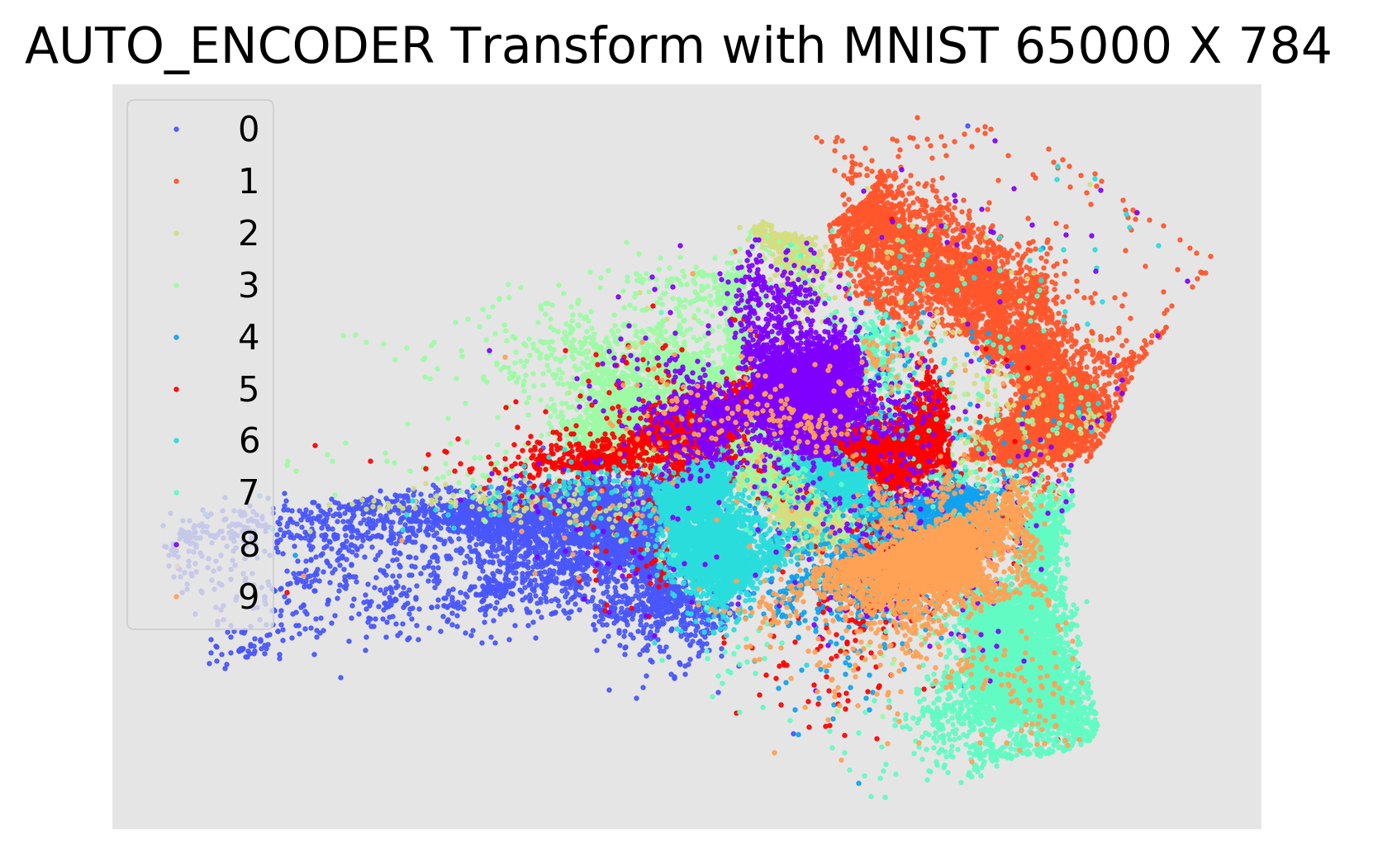5. 参考文档
PCA的数学原理 http://blog.codinglabs.org/articles/pca-tutorial.html浅谈流形学习 http://blog.pluskid.org/?p=533t-SNE完整笔记 http://www.datakit.cn/blog/2017/02/05/t_sne_full.html
展开全文• ## 降维

千次阅读 2019-06-13 20:25:12
可以发现，虽然是两个变量，但它们传达的信息是一致的，即物体的重量。所以我们只需选用其中的一个就能保留原始意义，把2维数据压缩到1维，这样的好处减少矩阵大小，在集合中就是减少维度，减少计算量，减少共线性。...
一、为什么要降维？
举个例子 两个特征“千克”，“磅”。可以发现，虽然是两个变量，但它们传达的信息是一致的，即物体的重量。所以我们只需选用其中的一个就能保留原始意义，把2维数据压缩到1维，这样的好处减少矩阵大小，在集合中就是减少维度，减少计算量，减少共线性。
在高维超正方体中，大多数点都分布在边界处： 在二维平面的一个正方形单元（一个 1×1 的正方形）中随机取一个点，那么随机选的点离所有边界大于 0.001（靠近中间位置）的概率为 0.4%（ 1 - 0.998^2 ）。在一个 1,0000 维的单位超正方体（一个 1×1×…×1 的立方体，有 10,000 个 1），这种可能性超过了 99.999999%。
高维数据集有很大风险分布的非常稀疏：在一个平方单位中随机选取两个点，那么这两个点之间的距离平均约为0.52，三维空间中这个距离是0.66，在一个 1,000,000 维超立方体中随机抽取两点，他们的距离大概是408.25。大多数训练实例可能彼此远离，训练集的维度越高，过拟合的风险就越大。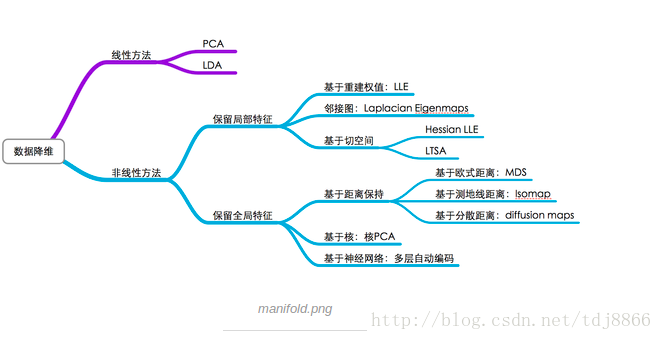二、降维技术
降低数据维度的方法主要有两种
仅保留原始数据中最相关的变量（特征选择）寻找一组较小的新变量，其中每个变量都是输入变量的组合，包含与输入变量基本相同的信息（降维）。
具体方法： 1、缺失值比率（Missing Value Ratio） 核心思想：空值率太低，果断舍弃（特征选择） code： train=pd.read_csv(“Train_UWu5bXk.csv”) #用.isnull().sum()检查每个变量中缺失值的占比 # 保存变量中的缺失值 a = train.isnull().sum()/len(train)*100
2、低方差滤波（Low Variance Filter） 核心思想：如果我们某一列的数值基本一致，也就是他的方差非常非常小，那么这个值没有价值，低方差变量携带的信息量很少，这是可以考虑删除 放在示例中，我们先估算缺失值： train[‘Item_Weight’].fillna(train[‘Item_Weight’].median, inplace=True)
train[‘Outlet_Size’].fillna(train[‘Outlet_Size’].mode(), inplace=True) 检查缺失值是否已经被填充： train.isnull().sum()/len(train)*100
再计算所有数值变量的方差： train.var() 再设定某个阈值，小于该阈值的全部删除
3、高相关滤波（High correlation filter） 核心思想：如果两个变量是高度相关的，它意味着他们具有相似的趋势，我们可以计算独立数值变量之间的相关性。如果相关性超过某个阈值，就删除其中一个变量 df=train.drop(‘Item_Outlet_Sales’, 1) df.corr() 如上表所示，示例数据集中不存在高相关变量，但通常情况下，如果一对变量之间的相关性大于0.5-0.6，那就应该考虑是否要删除一列了。
4、随机森林 random forest 随机森林是一种广泛使用的特征选择算法，他会自动计算各个特征之间的重要性，无需单独编码。（注意：在开始降维前，我们先把数据转换成数字格式，因为随机森林只接受数字）
5、反向特征消除（backward feature elimination）
先获取数据集n个变量，然后用他们训练一个模型计算模型性能在删除每个变量（n次）后计算模型性能，即我们每次都去掉一个变量，用剩余的n-1个变量训练模型。确定对模型性能影响最小的变量，把他删除重复此过程，知道不在能删除变量
lreg = LinearRegression() rfe = RFE(lreg, 10) rfe = rfe.fit_transform(df, train.Item_Outlet_Sales)
6、向前特征选择（Forward Feature selection） 前向特征选择其实就是反向特征消除的相反过程，即找到能改善模型性能的最佳特征，而不是删除弱影响特征。
选择一个特征，用每个特征训练模型n次，得到n个模型。  选择模型性能最佳的变量作为初始变量。  每次添加一个变量继续训练，重复上一过程，最后保留性能提升最大的变量。  一直添加，一直筛选，直到模型性能不再有明显提高。
7、因子分析（Factor Analysis） 因子分析是一种常见的统计方法，它能从多个变量中提取共性因子，并得到最优解。假设我们有两个变量：收入和教育。它们可能是高度相关的，因为总体来看，学历高的人一般收入也更高，反之亦然。所以它们可能存在一个潜在的共性因子，比如“能力”。
8、主成分分析 PCA 核心思想： 找到接近数据集分布的超平面，然后将所有的数据都投影到这个超平面上。 如果说因子分析是假设存在一系列潜在因子，能反映变量携带的信息，那PCA就是通过正交变换将原始的n维数据集变到一个新的被称作主成分的数据集中，即从现有的大量变量中提取一组新的变量。
主成分是原始变量的线性组合第一个主成分具有最大的方差值（选择保持最大方差的轴，因为它比其他投影损失更少的信息。）第二主成分试图解释数据集中剩余的方差，并且与第一主成分不相关（正交）第三主成分试图解释前两个主成分等没有解释的方差
9、独立分量分析（ICA）
总结： 缺失值比率：如果数据集的缺失值太多，我们可以用这种方法减少变量数。 低方差滤波：这个方法可以从数据集中识别和删除常量变量，方差小的变量对目标变量影响不大，所以可以放心删去。 高相关滤波：具有高相关性的一对变量会增加数据集中的多重共线性，所以用这种方法删去其中一个是有必要的。 随机森林：这是最常用的降维方法之一，它会明确算出数据集中每个特征的重要性。 前向特征选择和反向特征消除：这两种方法耗时较久，计算成本也都很高，所以只适用于输入变量较少的数据集。 因子分析：这种方法适合数据集中存在高度相关的变量集的情况。 PCA：这是处理线性数据最广泛使用的技术之一。 ICA：我们可以用ICA将数据转换为独立的分量，使用更少的分量来描述数据。 ISOMAP：适合非线性数据处理。 t-SNE：也适合非线性数据处理，相较上一种方法，这种方法的可视化更直接。 UMAP：适用于高维数据，与t-SNE相比，这种方法速度更快。
原文地址： www.analyticsvidhya.com/blog/2018/08/dimensionality-reduction-techniques-python/
展开全文特征工程
• ## 通俗理解PCA降维作用

万次阅读 多人点赞 2017-08-13 16:37:05
本文主要介绍一种降维方法，PCA（Principal Component Analysis，主成分分析）。...2. 降维可以在压缩数据的同时让信息损失最小化； 3. 理解几百个维度的数据结构很困难，两三个维度的数据通过可视化更容易理解。。
概述
本文主要介绍一种降维方法，PCA（Principal Component Analysis，主成分分析）。降维致力于解决三类问题：
降维可以缓解维度灾难问题；降维可以在压缩数据的同时让信息损失最小化；理解几百个维度的数据结构很困难，两三个维度的数据通过可视化更容易理解。
下面，将从简介、计算步骤、应用三方面进行理解PCA的降维作用。
PCA 简介
在理解特征提取与处理时，涉及高维特征向量的问题往往容易陷入维度灾难。随着数据集维度的增加，算法学习需要的样本数量呈指数级增加。有些应用中，遇到这样的大数据是非常不利的，而且从大数据集中学习需要更多的内存和处理能力。另外，随着维度的增加，数据的稀疏性会越来越高。在高维向量空间中探索同样的数据集比在同样稀疏的数据集中探索更加困难。
主成分分析 也称为 卡尔胡宁-勒夫变换（Karhunen-Loeve Transform），是一种用于探索高维数据结构的技术。PCA 通常用于高维数据集的探索与可视化；还可以用于数据压缩，数据预处理等。PCA 可以把可能具有相关性的高维变量合成线性无关的低维变量，称为主成分（ principal components）。新的低维数据集会尽可能的保留原始数据的变量。
PCA将数据投射到一个低维子空间实现降维。例如，二维数据集降维就是把点投射成一条线，数据集的每个样本都可以用一个值表示，不需要两个值。三维数据集可以降成二维，就是把变量映射成一个平面。一般情况下，

n

n

维数据集可以通过映射降成

k

k

维子空间，其中

k

≤

n

k{\le}n

。
假如你是一本养花工具宣传册的摄影师，你正在拍摄一个水壶。水壶是三维的，但是照片是二维的，为了更全面的把水壶展示给客户，你需要从不同角度拍几张图片。下图是你从四个方向拍的照片：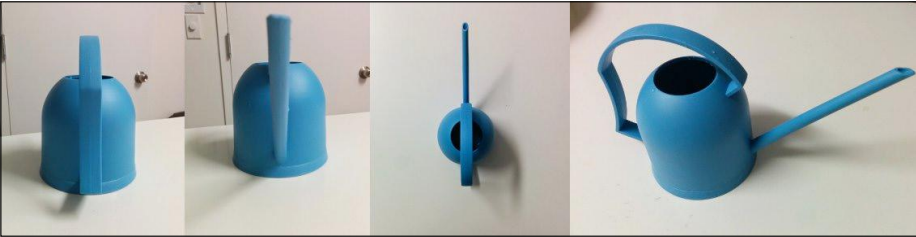第一张图里水壶的背面可以看到，但是看不到前面。第二张图是拍前面，可以看到壶嘴，这张图可以提供了第一张图缺失的信息，但是壶把看不到了。从第三张俯视图里无法看出壶的高度。第四张图是你真正想要的，水壶的高度，顶部，壶嘴和壶把都清晰可见。
PCA 的设计理念与此类似，它可以将高维数据集映射到低维空间的同时，尽可能的保留更多变量。PCA 旋转数据集与其主成分对齐，将最多的变量保留到第一主成分中。假设我们有下图所示的数据集：数据集看起来像一个从原点到右上角延伸的细长扁平的椭圆。要降低整个数据集的维度，我们必须把点映射成一条线。下图中的两条线都是数据集可以映射的，映射到哪条线样本变化最大？显然，样本映射到黑色虚线的变化比映射到红色点线的变化要大的多。实际上，这条黑色虚线就是第一主成分。第二主成分必须与第一主成分正交，也就是说第二主成分必须是在统计学上独立的，会出现在与第一主成分垂直的方向，如下图所示：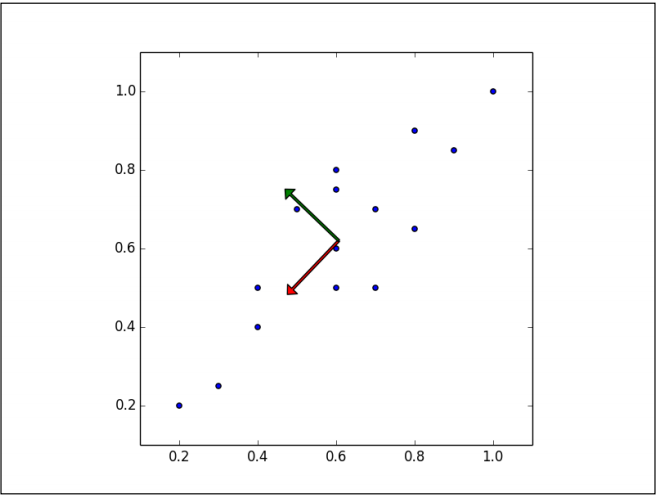后面的每个主成分也会尽量多的保留剩下的变量，唯一的要求就是每一个主成分需要和前面的主成分正交。
现在假设数据集是三维的，散点图看起来像是沿着一个轴旋转的圆盘。这些点可以通过旋转和变换使圆盘完全变成二维的。现在这些点看着像一个椭圆，第三维上基本没有变量，可以被忽略。
当数据集不同维度上的方差分布不均匀的时候，PCA最有用。（如果是一个球壳形数据集，PCA不能有效的发挥作用，因为各个方向上的方差都相等；没有丢失大量的信息维度一个都不能忽略）。
PCA的计算步骤
在介绍 PCA 的运行步骤之前，有一些术语需要说明一下。
方差，协方差和协方差矩阵
（对此概念不是很理解可以参考附录链接。）如何通俗易懂地解释「协方差」与「相关系数」的概念？中“GRAYLAMB”的回答。
方差（Variance） 是度量一组数据的分散程度。方差是各个样本与样本均值的差的平方和的均值：

s

2

=

∑

i

=

1

n

(

X

i

−

X

ˉ

)

2

n

−

1

s^2 = \frac {\sum_{i=1}^n {{(X_i-\bar X)}^2}} {n-1}

协方差（Covariance） 是度量两个变量的变动的同步程度，也就是度量两个变量线性相关性程度。如果两个变量的协方差为

0

0

，则统计学上认为二者线性无关。注意两个无关的变量并非完全独立，只是没有线性相关性而已。计算公式如下：

c

o

v

(

X

,

Y

)

=

∑

i

=

1

n

(

X

i

−

X

ˉ

)

(

Y

i

−

Y

ˉ

)

n

−

1

cov(X,Y)=\frac {\sum_{i=1}^n {(X_i-\bar X)(Y_i-\bar Y)}} {n-1}

如果协方差大于0表示一个变量增大是另一个变量也会增大，即正相关，协方差小于0表示一个变量增大是另一个变量会减小，即负相关。
协方差矩阵（Covariance matrix） 由数据集中两两变量的协方差组成。矩阵的第

(

i

,

j

)

(i,j)

个元素是数据集中第

i

i

和第

j

j

个元素的协方差。例如，三维数据的协方差矩阵如下所示：

C

=

[

c

o

v

(

x

1

,

x

1

)

c

o

v

(

x

1

,

x

2

)

c

o

v

(

x

1

,

x

3

)

c

o

v

(

x

2

,

x

1

)

c

o

v

(

x

2

,

x

2

)

c

o

v

(

x

2

,

x

3

)

c

o

v

(

x

3

,

x

1

)

c

o

v

(

x

3

,

x

2

)

c

o

v

(

x

3

,

x

3

)

]

C= \begin{bmatrix} cov(x_1,x_1) & cov(x_1,x_2) & cov(x_1,x_3)\\ cov(x_2,x_1) & cov(x_2,x_2) & cov(x_2,x_3)\\ cov(x_3,x_1) & cov(x_3,x_2) & cov(x_3,x_3)\\ \end{bmatrix}

让我们计算下表数据的协方差矩阵：

x

1

x

2

x

3

2

0

−

1.4

2.2

0.2

−

1.5

2.4

0.1

−

1

1.9

0

−

1.2

\begin{array} {ccc} x_1 && x_2 && x_3\\ \hline 2 && 0 && -1.4 \\ 2.2 && 0.2 && -1.5 \\ 2.4 && 0.1 && -1 \\ 1.9 && 0 && -1.2 \\ \end{array}

可以由 python 中的numpy包计算均值和协方差：
import numpy as np
X = [[2, 0, -1.4],
[2.2, 0.2, -1.5],
[2.4, 0.1, -1],
[1.9, 0, -1.2]]
print(np.mean(X,axis=0))
print(np.cov(np.array(X).T))

得到三个变量的样本均值分别是 2.125，0.075 和 -1.275；协方差矩阵为：

[

0.04916667

0.01416667

0.01916667

0.01416667

0.00916667

−

0.00583333

0.01916667

−

0.00583333

0.04916667

]

\begin{bmatrix} 0.04916667 & 0.01416667 & 0.01916667 \\ 0.01416667 & 0.00916667 & -0.00583333 \\ 0.01916667 & -0.00583333 & 0.04916667 \\ \end{bmatrix}

特征向量和特征值
（可以直观的理解：“特征向量是坐标轴，特征值是坐标”） 向量是具有 大小（magnitude） 和 方向（direction） 的几何概念。 特征向量（eigenvector） 是由满足如下公式的矩阵得到的一个非零向量：

A

ν

⃗

=

λ

ν

⃗

A \vec \nu = \lambda \vec \nu

其中，

ν

⃗

\vec \nu

是特征向量，

A

A

是方阵，

λ

\lambda

是特征值。经过

A

A

变换之后，特征向量的方向保持不变，只是其大小发生了特征值倍数的变化。也就是说，一个特征向量左乘一个矩阵之后等于等比例放缩（scaling）特征向量。德语单词 eigen 的意思是：“属于…或…专有（ belonging to or peculiar to）”；矩阵的特征向量是属于并描述数据集结构的向量。
特征向量和特征值只能由方阵得出，且并非所有方阵都有特征向量和特征值。如果一个矩阵有特征向量和特征值，那么它的每个维度都有一对特征向量和特征值。矩阵的主成分是由其协方差矩阵的特征向量，按照对应的特征值大小排序得到的。最大的特征值就是第一主成分，第二大的特征值就是第二主成分，以此类推。
让我们来计算下面矩阵的特征向量和特征值：

A

=

[

1

−

2

2

−

3

]

A=\begin{bmatrix}1 & -2 \\2 & -3 \\\end{bmatrix}

根据前面的公式

A

A

乘以特征向量，必然等于特征值乘以特征向量。我们建立特征方程求解：

(

A

−

λ

I

)

ν

⃗

=

0

(A- \lambda I) \vec \nu=0

∣

A

−

λ

∗

I

∣

=

∣

[

1

−

2

2

−

3

]

−

[

λ

0

0

λ

]

∣

=

0

|A-\lambda * I| = \begin{vmatrix}\begin{bmatrix}1 & -2 \\2 & -3 \\\end{bmatrix}-\begin{bmatrix}\lambda & 0 \\0 & \lambda \\\end{bmatrix}\end{vmatrix}= 0

从特征方程可以看出，矩阵与单位矩阵和特征值乘积的矩阵行列式为0，即：

∣

[

1

−

λ

−

2

2

−

3

−

λ

]

∣

=

(

λ

+

1

)

(

λ

+

1

)

=

0

\begin{vmatrix}\begin{bmatrix}1-\lambda & -2 \\2 & -3-\lambda\\\end{bmatrix}\end{vmatrix}=(\lambda+1)(\lambda+1)= 0

矩阵的两个特征值都等于-1。现在再用特征值来解特征向量。 把

λ

=

−

1

\lambda=-1

代入：

(

A

−

λ

)

ν

⃗

=

0

(A- \lambda ) \vec \nu=0

得：

(

[

1

−

2

2

−

3

]

−

[

λ

0

0

λ

]

)

ν

⃗

=

[

1

−

λ

−

2

2

−

3

−

λ

]

ν

⃗

=

[

1

−

λ

−

2

2

−

3

−

λ

]

[

ν

1

,

1

ν

1

,

2

]

=

[

1

−

(

−

1

)

−

2

2

−

3

−

(

−

1

)

]

[

ν

1

,

1

ν

1

,

2

]

=

[

2

−

2

2

−

2

]

[

ν

1

,

1

ν

1

,

2

]

=

0

\begin{aligned} \begin{pmatrix} \begin{bmatrix}1 & -2 \\ 2 & -3 \end{bmatrix} - \begin{bmatrix} \lambda & 0 \\ 0 & \lambda \end{bmatrix} \end{pmatrix}\vec\nu & = \begin{bmatrix} 1-\lambda & -2 \\ 2 & -3-\lambda \end{bmatrix}\vec\nu \\ & = \begin{bmatrix}1-\lambda & -2 \\2 & -3-\lambda \end{bmatrix} \begin{bmatrix}\nu_{1,1} \\ \nu_{1,2} \end{bmatrix} \\ & = \begin{bmatrix}1-(-1) & -2 \\ 2 & -3-(-1) \end{bmatrix} \begin{bmatrix}\nu_{1,1} \\ \nu_{1,2} \end{bmatrix} \\ & = \begin{bmatrix} 2 & -2 \\ 2 & -2 \end{bmatrix} \begin{bmatrix} \nu_{1,1} \\ \nu_{1,2} \end{bmatrix} \\ & = 0 \end{aligned}

所以有：

{

2

ν

1

,

1

−

(

2

ν

1

,

2

)

=

0

2

ν

1

,

1

−

(

2

ν

1

,

2

)

=

0

\begin{cases} 2\nu_{1,1} - (2\nu_{1,2})=0 \\ 2\nu_{1,1}-(2\nu_{1,2})=0 \end{cases}

任何满足方程

（

A

ν

⃗

=

λ

ν

⃗

）

（A \vec \nu = \lambda \vec \nu）

的非零向量（取

ν

⃗

=

[

1

1

]

\vec\nu=\begin{bmatrix}1\\\ 1\end{bmatrix}

）都可以作为特征向量：

[

1

−

2

2

−

3

]

[

1

1

]

=

[

−

1

−

1

]

=

−

1

×

[

1

1

]

\begin{bmatrix} 1 & -2 \\ 2 & -3 \end{bmatrix} \begin{bmatrix}1\\1\end{bmatrix}= \begin{bmatrix}-1\\-1\end{bmatrix}= -1×\begin{bmatrix}1\\ 1\end{bmatrix}

PCA需要单位特征向量，也就是

L

2

L_2

范数

∥

x

∥

=

x

1

2

+

x

2

2

+

⋯

+

x

n

2

\begin{Vmatrix}x \\\end{Vmatrix}=\sqrt {x_1^2 + x_2^2 + \dots + x_n^2}

等于1的特征向量。
于是有：

∥

[

1

1

]

∥

=

1

2

+

1

2

=

2

\begin{Vmatrix}\begin{bmatrix}1 \\1 \\\end{bmatrix}\end{Vmatrix}=\sqrt {1^2+1^2}=\sqrt 2

对应的单位特征向量是：

[

1

1

]

/

2

=

[

0.70710678

0.70710678

]

\begin{bmatrix} 1 \\ 1 \\ \end{bmatrix} / {\sqrt 2}=\begin{bmatrix}0.70710678 \\0.70710678 \\\end{bmatrix}

这里可以通过numpy检验手算的特征向量是否正确。eig 函数返回特征值和特征向量的元组：
import numpy as np
w, v = np.linalg.eig(np.array([[1, -2], [2, -3]]))
print('特征值：{}\n特征向量：{}'.format(w,v))

输出（这里特征值不同为1，是由于python编译器对浮点数据精度要求所致）：
特征值: [-0.99999998 -1.00000002]
特征向量: 	[[ 0.70710678  0.70710678]
[ 0.70710678  0.70710678]]

用PCA降维
让我们用 PCA 方法把下表二维数据降成一维：

x

1

x

2

0.9

1

2.4

2.6

1.2

1.7

0.5

0.7

0.3

0.7

1.8

1.4

0.5

0.6

0.3

0.6

2.5

2.6

1.3

1.1

\begin{array} {cc} x_1 && x_2 \\ \hline 0.9 && 1 \\ 2.4 && 2.6\\ 1.2 && 1.7\\ 0.5 && 0.7\\ 0.3 && 0.7\\ 1.8 && 1.4\\ 0.5 && 0.6\\ 0.3 && 0.6\\ 2.5 && 2.6\\ 1.3 && 1.1\\ \end{array}

PCA 第一步是用样本数据减去样本均值：

x

1

x

2

0.9

−

1.17

=

−

0.27

1

−

1.3

=

−

0.3

2.4

−

1.17

=

1.23

2.6

−

1.3

=

1.3

1.2

−

1.17

=

0.03

1.7

−

1.3

=

0.4

0.5

−

1.17

=

−

0.67

0.7

−

1.3

=

−

0.6

0.3

−

1.17

=

−

0.87

0.7

−

1.3

=

−

0.6

1.8

−

1.17

=

0.63

1.4

−

1.3

=

0.1

0.5

−

1.17

=

−

0.67

0.6

−

1.3

=

−

0.7

0.3

−

1.17

=

−

0.87

0.6

−

1.3

=

−

0.7

2.5

−

1.17

=

1.33

2.6

−

1.3

=

1.3

1.3

−

1.17

=

0.13

1.1

−

1.3

=

−

0.2

\begin{array}{cc} x_1 && x_2 \\ \hline 0.9-1.17=-0.27 && 1-1.3=-0.3 \\ 2.4-1.17=1.23 && 2.6-1.3=1.3 \\ 1.2-1.17=0.03 && 1.7-1.3=0.4 \\ 0.5-1.17=-0.67 && 0.7-1.3=-0.6 \\ 0.3-1.17=-0.87 && 0.7-1.3=-0.6 \\ 1.8-1.17=0.63 && 1.4-1.3=0.1 \\ 0.5-1.17=-0.67 && 0.6-1.3=-0.7 \\ 0.3-1.17=-0.87 && 0.6-1.3=-0.7 \\ 2.5-1.17=1.33 && 2.6-1.3=1.3 \\ 1.3-1.17=0.13 && 1.1-1.3=-0.2 \\ \end{array}

然后，我们计算数据的主成分。前面介绍过，矩阵的主成分是其协方差矩阵的特征向量按照对应的特征值大小排序得到的。主成分可以通过两种方法计算：第一种方法是计算数据协方差矩阵。因为协方差矩阵是方阵，所以我们可以用前面的方法计算特征值和特征向量。第二种方法是用数据矩阵的奇异值分解（singular value decomposition）来找协方差矩阵的特征向量和特征值的平方根。我们先介绍第一种方法，然后介绍scikit-learn的 PCA 实现，也就是第二种方法。上述数据集的解释变量协方差矩阵如下：

C

=

[

0.6867777778

0.6066666667

0.6066666667

0.5977777778

]

C=\begin{bmatrix} 0.6867777778 & 0.6066666667 \\0.6066666667 & 0.5977777778\\\end{bmatrix}

用前面介绍过的方法，特征值是1.25057433和0.03398123，单位特征向量是：

[

0.73251454

0.68075138

0.68075138

0.73251454

]

\begin{bmatrix}0.73251454 & 0.68075138 \\0.68075138 & 0.73251454 \\\end{bmatrix}

下面我们把数据映射到主成分上。第一主成分是最大特征值对应的特征向量，因此我们要建一个转换矩阵，它的每一列都是主成分的特征向量。如果我们要把5维数据降成3维，那么我们就要用一个3维矩阵做转换矩阵。在本例中，我们将把我们的二维数据映射成一维，因此我们只需要用特征向量中的第一主成分作为转换矩阵。最后，我们用数据矩阵右乘转换矩阵。下面就是第一主成分映射的结果：

[

−

0.27

−

0.3

1.23

1.3

0.03

0.4

−

0.67

0.6

−

0.87

0.6

0.63

0.1

−

0.67

−

0.7

−

0.87

−

0.7

1.33

1.3

0.13

−

0.2

]

[

0.73251454

0.68075138

]

=

[

−

0.40200434

1.78596968

0.29427599

−

0.08233391

−

0.22883682

0.5295593

−

0.96731071

−

1.11381362

1.85922113

−

0.04092339

]

\begin{bmatrix}-0.27 & -0.3 \\1.23 & 1.3 \\0.03 & 0.4 \\-0.67 & 0.6 \\-0.87 & 0.6 \\0.63 & 0.1 \\-0.67 & -0.7 \\-0.87 & -0.7 \\1.33 & 1.3 \\0.13 & -0.2\\\end{bmatrix} \begin{bmatrix}0.73251454\\0.68075138\\\end{bmatrix}= \begin{bmatrix}-0.40200434 \\ 1.78596968 \\ 0.29427599 \\-0.08233391 \\-0.22883682 \\ 0.5295593 \\-0.96731071 \\-1.11381362 \\ 1.85922113 \\-0.04092339 \\\end{bmatrix}

通过numpy包中的矩阵调用实现过程如下：
import numpy as np
x = np.mat([[ 0.9, 2.4, 1.2, 0.5, 0.3, 1.8, 0.5, 0.3, 2.5, 1.3],
[ 1, 2.6, 1.7, 0.7, 0.7, 1.4, 0.6, 0.6, 2.6, 1.1]])
x = x.T
T = x - x.mean(axis=0)
C = np.cov(x.T)
w,v = np.linalg.eig(C)
v_ = np.mat(v[:,0])		#每个特征值对应的是特征矩阵的每个列向量
v_ = v_.T		#默认以行向量保存，转换成公式中的列向量形式
y = T * v_
print(y)

其实到这可以结束了…
= = = = = = = = = = = = = = = =无耻分割线= = = = = = = = = = = = = = = = = =
PCA的运用
高维数据可视化
二维或三维数据更容易通过可视化发现模式。一个高维数据集是无法用图形表示的，但是我们可以通过降维方法把它降成二维或三维数据来可视化。
Fisher1936年收集了三种鸢尾花分别 50 个样本数据（Iris Data）：Setosa、Virginica、Versicolour。解释变量是花瓣（petals）和萼片（sepals）长度和宽度的测量值，响应变量是花的种类。鸢尾花数据集经常用于分类模型测试，scikit-learn中也有。让我们把iris数据集降成方便可视化的二维数据：
import matplotlib.pyplot as plt
from sklearn.decomposition import PCA

首先，我们导入鸢尾花数据集和PCA估计器。PCA类把主成分的数量作为超参数，和其他估计器一样，PCA也用fit_transform()返回降维的数据矩阵：
data = load_iris()
y = data.target
X = data.data
pca = PCA(n_components=2)
reduced_X = pca.fit_transform(X)

最后，我们把图形画出来：
red_x, red_y = [], []
blue_x, blue_y = [], []
green_x, green_y = [], []
for i in range(len(reduced_X)):
if y[i] == 0:
red_x.append(reduced_X[i])
red_y.append(reduced_X[i])
elif y[i] == 1:
blue_x.append(reduced_X[i])
blue_y.append(reduced_X[i])
else:
green_x.append(reduced_X[i])
green_y.append(reduced_X[i])
plt.scatter(red_x, red_y, c='r', marker='x')
plt.scatter(blue_x, blue_y, c='b', marker='D')
plt.scatter(green_x, green_y, c='g', marker='.')
plt.show()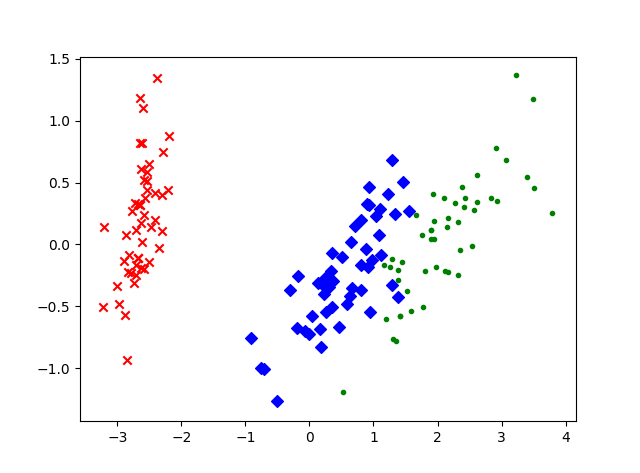降维的数据如上图所示。每个数据集中三个类都用不同的符号标记。从这个二维数据图中可以明显看出，有一个类与其他两个重叠的类完全分离。这个结果可以帮助我们选择分类模型。
脸部识别
现在让我们用 PCA 来解决一个脸部识别问题。脸部识别是一个监督分类任务，用于从照片中认出某个人。本例中，我们用剑桥大学 AT&T 实验室的 Faces数据集，这个数据集包含 40 个人每个人 10 张照片。这些照片是在不同的光照条件下拍摄的，每张照片的表情也不同。照片都是黑白的，尺寸为 92 x 112 像素。虽然这些图片都不大，但是每张图片的按像素强度排列的特征向量也有（92 x 112=）10304 维。这些高维数据的训练可能需要很多样本才能避免拟合过度。而我们样本量并不大，所有我们用 PCA 计算一些主成分来表示这些照片。
我们可以把照片的像素强度矩阵转换成向量，然后用所有的训练照片的向量建一个矩阵。每个照片都是数据集主成分的线性组合。在脸部识别理论中，这些主成分称为特征脸（eigenfaces）。特征脸可以看成是脸部的标准化组成部分。数据集中的每张脸都可以通过一些标准脸的组合生成出来，或者说是最重要的特征脸线性组合的近似值。
from os import walk, path
import numpy as np
import mahotas as mh
from sklearn.cross_validation import train_test_split
from sklearn.cross_validation import cross_val_score
from sklearn.preprocessing import scale
from sklearn.decomposition import PCA
from sklearn.linear_model import LogisticRegression
from sklearn.metrics import classification_report
X = []
y = []

下面我们把照片导入Numpy数组，然后把它们的像素矩阵转换成向量：
for dir_path, dir_names, file_names in walk('C:/Users/HLB/Desktop/first blog/att_faces/'):
#walk() 函数内存放的是数据的绝对路径，同时注意斜杠的方向。
for fn in file_names:
if fn[-3:] == 'pgm':
image_filename = path.join(dir_path, fn)
y.append(dir_path)
X = np.array(X)

然后，我们用交叉检验建立训练集和测试集，在训练集上用PCA：
X_train, X_test, y_train, y_test = train_test_split(X, y)
pca = PCA(n_components=150)

我们把所有样本降到150维，然后训练一个逻辑回归分类器。数据集包括40个类；scikit-learn底层会自动用 one versus all 策略创建二元分类器：
X_train_reduced = pca.fit_transform(X_train)
X_test_reduced = pca.transform(X_test)
print('训练集数据的原始维度是：{}'.format(X_train.shape))
print('PCA降维后训练集数据是：{}'.format(X_train_reduced.shape))
classifier = LogisticRegression()
accuracies = cross_val_score(classifier, X_train_reduced, y_train)

训练集数据的原始维度是：(300, 10304) PCA降维后训练集数据是：(300, 150)
最后，我们用交叉验证和测试集评估分类器的性能。分类器的平均综合评价指标（F1 score）是0.88，但是需要花费更多的时间训练，在更多训练实例的应用中可能会更慢。
print('交叉验证准确率是：{}\n{}'.format(np.mean(accuracies), accuracies))
classifier.fit(X_train_reduced, y_train)
predictions = classifier.predict(X_test_reduced)
print(classification_report(y_test, predictions))

最终的分析结果：
交叉验证准确率是：0.829757290513
[ 0.83035714  0.83333333  0.8255814 ]
precision    recall  f1-score   support
C:/Users/HLB/Desktop/first blog/att_faces/s1		1.00      1.00      1.00         1
C:/Users/HLB/Desktop/first blog/att_faces/s10       1.00      1.00      1.00         2
C:/Users/HLB/Desktop/first blog/att_faces/s11       1.00      1.00      1.00         2
C:/Users/HLB/Desktop/first blog/att_faces/s12       1.00      1.00      1.00         2
C:/Users/HLB/Desktop/first blog/att_faces/s13       1.00      1.00      1.00         4
C:/Users/HLB/Desktop/first blog/att_faces/s14       1.00      1.00      1.00         3
C:/Users/HLB/Desktop/first blog/att_faces/s15       1.00      1.00      1.00         2
C:/Users/HLB/Desktop/first blog/att_faces/s16       1.00      0.75      0.86         4
C:/Users/HLB/Desktop/first blog/att_faces/s17       1.00      1.00      1.00         4
C:/Users/HLB/Desktop/first blog/att_faces/s18       1.00      1.00      1.00         3
C:/Users/HLB/Desktop/first blog/att_faces/s19       1.00      1.00      1.00         3
C:/Users/HLB/Desktop/first blog/att_faces/s2       	1.00      1.00      1.00         2
C:/Users/HLB/Desktop/first blog/att_faces/s20       1.00      1.00      1.00         2
C:/Users/HLB/Desktop/first blog/att_faces/s21       1.00      1.00      1.00         2
C:/Users/HLB/Desktop/first blog/att_faces/s22       1.00      1.00      1.00         2
C:/Users/HLB/Desktop/first blog/att_faces/s23       1.00      1.00      1.00         4
C:/Users/HLB/Desktop/first blog/att_faces/s24       1.00      1.00      1.00         2
C:/Users/HLB/Desktop/first blog/att_faces/s25       1.00      1.00      1.00         4
C:/Users/HLB/Desktop/first blog/att_faces/s26       1.00      1.00      1.00         5
C:/Users/HLB/Desktop/first blog/att_faces/s27       0.50      1.00      0.67         1
C:/Users/HLB/Desktop/first blog/att_faces/s28       1.00      0.67      0.80         3
C:/Users/HLB/Desktop/first blog/att_faces/s29       1.00      1.00      1.00         2
C:/Users/HLB/Desktop/first blog/att_faces/s3      	1.00      1.00      1.00         1
C:/Users/HLB/Desktop/first blog/att_faces/s30       1.00      1.00      1.00         3
C:/Users/HLB/Desktop/first blog/att_faces/s31       1.00      1.00      1.00         3
C:/Users/HLB/Desktop/first blog/att_faces/s32       1.00      1.00      1.00         1
C:/Users/HLB/Desktop/first blog/att_faces/s33       1.00      1.00      1.00         1
C:/Users/HLB/Desktop/first blog/att_faces/s34       1.00      1.00      1.00         3
C:/Users/HLB/Desktop/first blog/att_faces/s35       1.00      1.00      1.00         2
C:/Users/HLB/Desktop/first blog/att_faces/s36       0.67      1.00      0.80         2
C:/Users/HLB/Desktop/first blog/att_faces/s37       0.50      1.00      0.67         1
C:/Users/HLB/Desktop/first blog/att_faces/s38       1.00      1.00      1.00         5
C:/Users/HLB/Desktop/first blog/att_faces/s39       1.00      1.00      1.00         3
C:/Users/HLB/Desktop/first blog/att_faces/s4      	1.00      1.00      1.00         1
C:/Users/HLB/Desktop/first blog/att_faces/s40       1.00      1.00      1.00         1
C:/Users/HLB/Desktop/first blog/att_faces/s5       	1.00      0.83      0.91         6
C:/Users/HLB/Desktop/first blog/att_faces/s6       	1.00      1.00      1.00         3
C:/Users/HLB/Desktop/first blog/att_faces/s7       	1.00      1.00      1.00         2
C:/Users/HLB/Desktop/first blog/att_faces/s8       	1.00      1.00      1.00         2
C:/Users/HLB/Desktop/first blog/att_faces/s9       	1.00      1.00      1.00         1
avg / total       0.98      0.97      0.97       100

总结
本文主要介绍 PCA 降维问题。高维数据不能轻易可视化。估计器训练高维数据集时，也可能出现维度灾难。通过主成分分析法缓解这些问题，将可能解释变量具有相关性的高维数据集，通过将数据映射到一个低维子空间，降维成一个线性无关的低维数据集。最后拓展用PCA将四维的鸢尾花数据集降成二维数据进行可视化；并将PCA用在一个脸部识别系统。
扩展阅读
如何通俗易懂地解释「协方差」与「相关系数」的概念？线性代数中，特征值与特征向量在代数和几何层面的实际意义是什么？7-dimensionality-reduction-with-pca机器学习/周志华著.–北京：清华大学出版署，2016（2017.2重印）ISBN 978-302-42328-7
展开全文python PCA 数据分析 数据挖掘
•研究论文
• 与主成分分析（PCA）算法相比，实验结果表明用该算法对数据集降维后，得到的属性约简集合的属性个数较多，K-means算法根据属性集合进行聚类的精度较高。实验结果证明该算法能有效地应用于信息表的属性约简方面。聚类 邻域粗糙集 主成分分析
• 为了提高降维的效果, 根据近邻点的选取对diffusion maps的降维效果影响, 利用数据近邻点分布的不同, 挖掘该数据点局部的密度信息, 能够更好地保持数据的流形结构。利用样本点聚类后的类别信息构造密度信息指数, 提出...流形学习 聚类 扩散映射
• 针对文本分类中信息增益降维方法的不足，提出了一种基于相对文档频的平衡信息增益（RDFBIG）降维方法。实验结果表明，RDFBIG能有效消除不同类别之间语料规模对分类精度的影响，取得了较好的分类效果。
• ## 降维相关

千次阅读 2017-12-04 22:54:09
降维相关降维相关 什么是降维 PCA LDA LLE LE 什么是降维一般来说，在ml里面，需要feature。而对于feature，我们又通常使用向量来表示。...直观地说，就是要既能降低维度，又能使得损失的信息尽量少。举个例子，如果


什么是降维PCALDALLELESNET-SNE

什么是降维
一般来说，在ml里面，需要feature。而对于feature，我们又通常使用向量来表示。所以，简单地说，降维就是将一个高维的向量映射为一个低维的向量。形象地说，降维可以看作一个函数，输入是一个D为的向量，输出是一个M维的向量。
那怎么样才算是一个好的降维结果呢？直观地说，就是要既能降低维度，又能使得损失的信息尽量少。举个例子，如果现在有淘宝店铺的特征，有非常多维，我们想降维，那要怎么做呢？假设特征中有两维特征是“浏览量”和“访客数”，其实这两者之间应该是具有强相关性的，直觉上删除其中一个并不会造成多大的信息损失。以上就是一个朴素的降维思想。而按照机器学习的方法，我们需要定义一个目标函数，并进行最优化。而不同的优化目标也就导致了不同的降维算法。
首先，来看看最直接的损失函数，reconstuction error：

1N∑i=1N||xi−xi~||2

\frac{1}{N} \sum^N_{i=1} ||x_i - \tilde{x_i}||^2

其中

xi~
$\tilde{x_i}$是

xi
$x_i$降维后得到的低维向量再次“升维”而还原出来的高维向量。上面的式子无脑符合“既能降低维度，又能使得损失的信息尽量少”这句话。虽然这种损失函数很直接，但缺点是不仅要想出降维的方法还要有还原的方法。
另一种损失函数是variance：

argmaxf1N∑i=1N(f(xi)−f(xi)¯)2注意那个横线覆盖整个f(xi)

arg max_f \frac{1}{N} \sum^N_{i=1} (f(x_i) - \bar{f(x_i)})^2 \\
注意那个横线覆盖整个f(x_i)

这里variance的意思是“使得特征具有最好的区分能力”，在式子中的表现就是方差了。
好了，下面开始从一个个具体的降维算法入手，讲述其中的一些数学原理。

PCA
PCA作为学术界和工业界都最为常见的一个降维算法，肯定是排第一个啦。
在真正讲PCA之前，先明确一下协方差和协方差矩阵。协方差

cov(x,y)=1n−1∑i=1nxiyi

cov(x,y) = \frac{1}{n-1} \sum_{i=1}^n x_i y_i

是为了检测x和y两个变量之间的相关性，为正表明正相关，且越大说明越正相关；反之则是负相关。单单协方差只能衡量两个变量，也就是说只能处理特征只有二维的情况。如果特征有多维，那么就是协方差矩阵出场的时候了:

C=⎛⎝⎜cov(x,x)cov(y,x)cov(z,x)cov(x,y)cov(y,y)cov(z,y)cov(x,z)cov(y,z)cov(z,z)⎞⎠⎟对于矩阵XM∗N，其协方差矩阵为CM∗M=1N−1XXT

C = \left(
\begin{array}{ccc}
cov(x,x) & cov(x,y) & cov(x,z) \\
cov(y,x) & cov(y,y) & cov(y,z) \\
cov(z,x) & cov(z,y) & cov(z,z) \\
\end{array}
\right)\\
对于矩阵X_{M*N}，其协方差矩阵为C_{M*M} = \frac{1}{N-1} X X^T

对角线上是方差，而其他位置是协方差。另外，我们有以下推导：

∵协方差的性质cov(x,y)=cov(y,x)∴上面的协方差矩阵就是一个实对称阵∴可以进行正交对角化，即存在正交矩阵U，使得UTΣU=Λ其中，Λ是一个对角矩阵，且对角线是矩阵Σ的特征值矩阵U是矩阵Σ的特征向量正交化得到的∴这是一个半正定矩阵（注意，光一个实对称阵是推不出半正定的，还要求特征值全大于等于零，可能还要求严格对角最优）所谓半正定矩阵，就是有XTMX≥0，其中X为向量∴可以进行Cholesky分解，即Σ=UTΛU，其中U是上三角矩阵，Λ是对角线元素都非负的对角矩阵∴Σ=UTΛU=[UTΛ1/2][Λ1/2U]=[Λ1/2U]T[Λ1/2U]∴Σ=CTC，其中C=Λ1/2

\because 协方差的性质cov(x,y) = cov(y,x) \\
\therefore 上面的协方差矩阵就是一个实对称阵 \\
\therefore 可以进行正交对角化，即存在正交矩阵U，使得 U^T \Sigma U = \Lambda \\
其中，\Lambda 是一个对角矩阵，且对角线是矩阵\Sigma 的特征值\\
矩阵U是矩阵\Sigma的特征向量正交化得到的\\
\therefore 这是一个半正定矩阵\\
（注意，光一个实对称阵是推不出半正定的，还要求特征值全大于等于零，可能还要求严格对角最优）\\
所谓半正定矩阵，就是有 X^T M X \geq 0，其中X为向量\\
\therefore 可以进行Cholesky分解，即 \\
\Sigma = U^T \Lambda U，其中U是上三角矩阵，\Lambda 是对角线元素都非负的对角矩阵 \\
\therefore \Sigma = U^T \Lambda U = [U^T \Lambda^{1/2}] [\Lambda^{1/2} U] = [\Lambda^{1/2} U]^T [\Lambda^{1/2} U]
\therefore \Sigma = C^T C，其中C = \Lambda^{1/2}

好了，协方差矩阵的基础讲完了，下面开始介绍PCA。
PCA的目的是将D维的向量降为M维（0< M< D）。有两个目标，一个是保留区分能力最强的M个特征，另一个是这M维特征之间相关性尽量小。前者体现在每一维特征自己的方差都较大，后者体现在不同维度特征的协方差较小。所以，从这两点出发，很自然地就想到了协方差矩阵。
设原始数据矩阵

XM∗N
$X_{M*N}$(M表示特征维数，N表示数据量)的协方差矩阵为

CM∗M
$C_{M*M}$，而我们想将X降维成

YK∗N
$Y_{K*N}$，也就是寻找一种线性变换，或者说一个矩阵P，使得

Y=PX
$Y=PX$。我们设Y的协方差矩阵为

DK∗K
$D_{K*K}$。根据上一段，我们希望这个矩阵D是一个对角矩阵，且对角线上的值越大越好。
那么，要怎么求P，才能使得这个D能满足我们的要求呢？我们来推导一下：

D=1N−1YYT=1N−1(PX)(PX)T=1N−1PXXTPT=P(1N−1XXT)PT=PCPT

D = \frac{1}{N-1} Y Y^T \\
= \frac{1}{N-1} (PX) (PX)^T \\
= \frac{1}{N-1} PX X^T P^T \\
= P (\frac{1}{N-1} X X^T) P^T \\
= P C P^T

所以，我们要找的P能使得原始的协方差矩阵对角化。形式化描述就是：求矩阵

S
$S$，使得SCST$S C S^T$是一个对角矩阵，并且对角元素从大到小排列。然后我们只需要取矩阵S的前K行组成的矩阵P，即可。接下来就是数学的东西了，上面介绍协方差矩阵的特性的时候提过了，不再多说。
PCA的一张二维降一维的图，这里有PCA的例子
PCA也有一些限制，它可以解决特征之间存在线性相关的情况，但对于非线性相关的效果就不怎么样了。另外，下面是我从网站粘下来的优缺点：
优点：  1. 以方差衡量信息的无监督学习，不受样本标签限制。  2. 各主成分之间正交，可消除原始数据成分间的相互影响。  3. 可减少指标选择的工作量。  4. 用少数指标代替多数指标，利用PCA降维是最常用的算法。  5. 计算方法简单，易于在计算机上实现。
缺点：  1. 主成分解释其含义往往具有一定的模糊性，不如原始样本完整。  2. 贡献率小的主成分往往可能含有对样本差异的重要信息。  3. 特征值矩阵的正交向量空间是否唯一有待讨论。  4. 无监督学习。

LDA
下面讲LDA，毕竟LDA与PCA是我们最常听说的两个降维算法。
LDA首先是一个有监督的降维算法，会利用类别信息。所以其核心思想就是“将高维的向量投影到低维的空间，使得投影后同一类点之间的距离较小，不同类之间距离较大”，也就是“最大化类间距离，最小化类内距离”。具体的，这个距离是通过方差来衡量的。
循序渐进，我们首先来看一下二分类的LDA。
注：说到二类LDA，那就是投影到一条直线上，也就是说，降维成一维。可能有人会问，在二类的前提下，如果我们现在想将一个D维的向量x降维成M维的向量y，应该怎么办呢？这时候可以这么回答，LDA对于二类的情况能降成一维，明显一维的情况足以区分二类（另外，LDA降维后的维数不能大于类别数减一，二类的最大只能降成一维）。所以LDA也可以看成是一个分类算法。
二类LDA过程如下：

假设样本原始的坐标为一个向量x投影后样本的坐标为：y=wTx，这里y就是一个标量，一个值类别1的中心点为：μ1=1N1∑x∈D1x类别2的中心点为：μ2=1N2∑x∈D2x类别1投影后的中心点为：μ¯1=1N1∑x∈D1wTx=wTμ1类别2投影后的中心点为：μ¯2=1N2∑x∈D2wTx=wTμ2我们使用两类中心点之间的距离来衡量类间距离“最大化类间距离”就是：max||μ¯1−μ¯2||2类别1投影后的类内距离：s¯21=∑y∈D1||y−μ¯1||2类别2投影后的类内距离：s¯22=∑y∈D2||y−μ¯2||2“最小化类内距离”就是：min(s¯21+s¯22)最终目的就是:J(w)=max||μ¯1−μ¯2||2s¯21+s¯22

假设样本原始的坐标为一个向量x\\
投影后样本的坐标为：y = w^T x，这里y就是一个标量，一个值 \\
类别1的中心点为：\mu_1 = \frac{1}{N_1} \sum_{x \in D_1} x \\
类别2的中心点为：\mu_2 = \frac{1}{N_2} \sum_{x \in D_2} x \\
类别1投影后的中心点为：\bar \mu_1 = \frac{1}{N_1} \sum_{x \in D_1} w^T x = w^T \mu_1\\
类别2投影后的中心点为：\bar \mu_2 = \frac{1}{N_2} \sum_{x \in D_2} w^T x = w^T \mu_2\\
我们使用两类中心点之间的距离来衡量类间距离 \\
“最大化类间距离”就是：\max ||\bar \mu_1 - \bar \mu_2||^2 \\
类别1投影后的类内距离：\bar s_1^2 = \sum_{y \in D_1} ||y - \bar \mu_1||^2 \\
类别2投影后的类内距离：\bar s_2^2 = \sum_{y \in D_2} ||y - \bar \mu_2||^2 \\
“最小化类内距离”就是：\min (\bar s_1^2 + \bar s_2^2) \\
最终目的就是: J(w) = \max \frac{||\bar \mu_1 - \bar \mu_2||^2}{\bar s_1^2 + \bar s_2^2} \\

接下来我们要稍作推导了：

||μ¯1−μ¯2||2=wT(μ1−μ2)(μ1−μ2)Tw=wTSBws¯21=∑y∈D1||y−μ¯1||2=∑x∈D1||wTx−wTμ1||2=∑x∈D1wT(x−μ1)(x−μ1)Tw=wTS1w则s¯21+s¯22=wTS1w+wTS2w=wTSWw所以有J(w)=wTSBwwTSWw，其中SB是类间散度矩阵，SW是类内散度矩阵SB=(μ1−μ2)(μ1−μ2)TSW=(x1−μ1)(x1−μ1)T+(x2−μ2)(x2−μ2)T接下来就是数学问题了，篇幅有限，不多做解释了。

||\bar \mu_1 - \bar \mu_2||^2 = w^T (\mu_1 - \mu_2) (\mu_1 - \mu_2)^T w = w^T S_B w \\
\bar s_1^2 = \sum_{y \in D_1} ||y - \bar \mu_1||^2 = \sum_{x \in D_1} ||w^T x - w^T \mu_1||^2 = \sum_{x \in D_1} w^T (x - \mu_1 )(x - \mu_1 )^T w = w^T S_1 w\\
则 \bar s_1^2 + \bar s_2^2 = w^T S_1 w + w^T S_2 w = w^T S_W w\\
所以有 J(w) = \frac{w^T S_B w}{w^T S_W w}，其中S_B是类间散度矩阵，S_W是类内散度矩阵\\
S_B =  (\mu_1 - \mu_2) (\mu_1 - \mu_2)^T \\
S_W = (x_1 - \mu_1 )(x_1 - \mu_1 )^T + (x_2 - \mu_2 )(x_2 - \mu_2 )^T \\
接下来就是数学问题了，篇幅有限，不多做解释了。

上面是二类的做法，那多类要怎么做呢（记住LDA降维后的维数不能大于类别数减一）？

首先，y=wTx还是同样适用，只不过这里y是一个向量，w是一个矩阵。对于类内散度矩阵，还是一样的配方，只不过相加的项数会变多，毕竟还是算每个点到中心点的距离。而对于类间散度矩阵确不能这么求了，因为有多个类别。所以我们定义一个全局散度矩阵，用来求每一个类别的中心点到总中心点的距离把这些距离求和，再求最大值SB=∑i=1NNi(μi−μ)(μi−μ)TSW=∑i=1N∑x∈Di(x−μi)(x−μi)T下面还是数学内容，不多赘述。

首先，y = w^T x 还是同样适用，只不过这里y是一个向量，w是一个矩阵。\\
对于类内散度矩阵，还是一样的配方，只不过相加的项数会变多，毕竟还是算每个点到中心点的距离。\\
而对于类间散度矩阵确不能这么求了，因为有多个类别。\\
所以我们定义一个全局散度矩阵，用来求每一个类别的中心点到总中心点的距离\\
把这些距离求和，再求最大值\\
S_B = \sum_{i=1}^N N_i (\mu_i - \mu) (\mu_i - \mu)^T \\
S_W = \sum_{i=1}^N \sum_{x \in D_i} (x - \mu_i) (x - \mu_i)^T \\
下面还是数学内容，不多赘述。

好了，关于LDA就说这么多。另外，关于“LDA降维后的维数不能大于类别数减一”与矩阵的秩，特征向量有关。具体为什么建议去看LDA接下来的数学推导，可以看这里。关于PCA和LDA的对比，可以看这里

LLE
上面的两种算法都是线性的，那对于非线性的情况要怎么处理呢？这里介绍一下LLE算法。
LLE算法的介绍可见Arrow Luo的局部线性嵌入降维算法的pdf版，这里就不介绍详细的算法步骤了，只提几个关键点帮助理解。
LLE应对的是非线性的情况（这里的非线性指的是无法线性映射到低维空间）。当然也不是所有的非线性情况都行，LLE有一个比较强的假设：原始数据满足流形，并且局部线性。LLE非线性，所以不能求一个矩阵来直接表示降维过程。LLE中是直接求高维向量在低维空间中的表示的。在求局部线性重构矩阵W的时候，在pdf的公式(4)中，X-WZ可以转化为WX-WZ的原因是

∑W=1
$\sum W = 1$。局部线性重构矩阵W的具体样子应该是这样的：总的应该是n*n的，n表示总节点数。每一行

Wi
$W_i$对应某一个节点i，然后这一行只有k个位置有非零值，表示节点i的k个近邻点。然后这一行其他位置都是0. 在求解低维空间中的向量y的时候加入了两个约束条件，目的是使得解唯一并且易于求解。不然的话就一个那个求最小值的式子，只知道一个W，解肯定不唯一。
LLE的优缺点可以看这篇博客

LE
LE算法和LLE算法一样是基于流行假设的。
设

Yi
$Y_i$为

Xi
$X_i$降维后的样本点，令矩阵

W
$W$表示节点之间的连接关系。则LE的思想就是最小化：

∑i=1,j=1NWij||yi−yj||2
\sum_{i=1,j=1}^N W_{ij} ||y_i - y_j ||^2

LE中文叫拉普拉斯特征映射，为什么要叫拉普拉斯呢？因为上面式子的求解需要运用到拉普拉斯矩阵，具体的推导过程如下：

定义对角矩阵D，Dii=∑j=1NWij令矩阵L=D−W，L即为拉普拉斯矩阵，为什么要L因为L是半正定矩阵，最小特征值是0，每一行的和均为0。这些特质方面代数计算min∑i=1,j=1NWij||yi−yj||2=mintr(YTLY)s.t.YTDY=I

定义对角矩阵D，D_{ii} = \sum_{j=1}^N W_{ij} \\
令矩阵L = D-W，L即为拉普拉斯矩阵，为什么要L\\
因为L是半正定矩阵，最小特征值是0，每一行的和均为0。这些特质方面代数计算\\
\min \sum_{i=1,j=1}^N W_{ij} ||y_i - y_j ||^2 = \min tr(Y^T L Y) \\
s.t. Y^T D Y = I

说到这里，我想说一下为什么LE和LLE要基于流形假设（只是我个人理解）。首先，流形的定义就不多说了，在这里可以看作一个不闭合的曲面。然后对于PCA和LDA这种线性降维算法，他们认为高维的数据是低维数据的一个线性映射。所以降维也就是找高维到低维的一个线性映射。而对于LE和LLE这种非线性算法，认为高维的数据是低维数据在高维空间中被扭曲形成的，这是一种非线性的映射。因为是非线性的，所以找不到线性的映射矩阵。另外，流形在局部可以近似认为是线性的（LLE），或者说在局部可以建立边连接（意思是在高维空间局部建立边连接，实际上在低维空间中这条边也是存在的）（LE），这就是为什么LE和LLE要基于流形假设。

SNE
算法思想是：在高维空间相似的数据点，映射到低维空间也是相似的。
设

Xi
$X_i$与

Xj
$X_j$为高维空间中的两个样本点，使用概率

pi|j
$p_i|j$来衡量两者的相似度。  设

Yi
$Y_i$与

Yj
$Y_j$为高维空间中的两个样本点，使用概率

qi|j
$q_i|j$来衡量两者的相似度。
形成两个概率分布P和Q，目的是最小化：

C=∑iKL(Pi||Qi)=∑i∑jpj|ilogpj|iqj|i

C=\sum_{i}KL(P_i||Q_i)=\sum_i \sum_j p_{j|i} \log \frac{p_{j|i}}{q_{j|i}}

那么概率具体应该怎么表示呢？正比于以x_i为中心的高斯分布的概率密度:

pj|i=exp(−∥∥xi−xj∥∥2/2σ2i)∑k≠iexp(−∥xi−xk∥2/2σ2i)表示xi以条件概率pj|i选择xj作为其邻近点

p_{j|i}=\frac{\exp (-\left \| x_i-x_j \right \|^2/2 \sigma_{i}^2)}{\sum_{k \neq i}\exp (-\left \| x_i-x_k \right \|^2/2 \sigma_{i}^2)}\\
表示x_i以条件概率p_{j|i}选择x_j作为其邻近点

低维空间中，正比于以y_i为中心的高斯分布的概率密度:

qj|i=exp(−∥∥yi−yj∥∥2)∑k≠iexp(−∥yi−yk∥2)

q_{j|i}=\frac{\exp (-\left \| y_i-y_j \right \|^2)}{\sum_{k \neq i}\exp (-\left \| y_i-y_k \right \|^2)}

为什么这么做可能是方便后面的计算，也有可能是因为后面迭代计算时加入了高斯噪声。
当然，考虑到对称性，最终定义:

pij=pji=pj|i+pi|j2

p_{ij}=p_{ji}=\frac{p_{j|i}+p_{i|j}}{2}

T-SNE
相对SNE就是将高斯分布改成了t-分布。
从正态总体中抽取容量为

N
$N$的随机样本，若该正态总体的均值为μ$\mu$，方差为

σ2
${\sigma}^2$。随机样本均值为

x¯
$\bar{x}$，方差为

s2=1N−1∑Ni=1(xi−x¯)2
$s^2=\frac{1}{N-1}\sum_{i=1}^{N}(x_i-\bar{x})^2$，随机变量

t
$t$可表示为：

t=x¯−μs/N−−√
t=\frac{\bar{x}-\mu}{s/\sqrt{N}}

原因是高斯分布的尾部较低，对异常点比较敏感。相比之下，tt分布的尾部较高，对异常点不敏感，保证了其鲁棒性，因此其拟合结果更为合理，较好的捕获了数据的整体特征。
展开全文• 在实际的生产和应用中，降维在一定的信息损失范围内，可以为我们节省大量的时间和成本。降维也成为了应用非常广泛的数据预处理方法。　降维具有如下一些优点：（1）使得数据集更易使用（2）降低算法的计算开销（3）...PCA
•研究论文
• 通过对栈式自编码器深度学习算法进行研究,提出一种深度学习降维信息损失度量方法,将香农信息理论运用到降维信息损失度量中,计算深度学习降维过程中信息损失量,并研究其与算法性能的关系,为深度学习算法的改进提供...深度学习
• 目录 一、相关背景 二、降维方法 1. PCA降维 1.1 PCA实例 1.2 代码实现(python+numpy) ...在许多领域的研究与应用中，通常需要对含有多个...多变量大数据集无疑会为研究和应用提供丰富的信息，但是也在一定程度上...数据 机器学习
• 降维方法 1：Multidimensional Scaling(MDS) MDS是一种降维或者可视化算法，通过使得降维之后的数据能够保留原始数据之间的相似度（或者不相似度，距离）等等，来将数据映射到低维空间。 假设原始数据的距离矩阵D...PCA MDS
• ## FDA降维

千次阅读 2016-03-24 10:53:19
LPP通过保持数据的局部结构获得很好的降维效果，但他只能用于无监督的情况，不能将样本的标签信息考虑在内。 由于类间散布矩阵不是满秩的，所以FDA只能将数据映射到维数小于类个数的低维空间，这是FDA的局限。...